This tutorial has been forked from awesome classes developed by Adam Wilson here: http://adamwilson.us/RDataScience/

The R Script associated with this page is available here. Download this file and open it (or copy-paste into a new script) with RStudio so you can follow along.

## 0.1 Data

In this module, we’ll primarily use the `mtcars` data object. The data was extracted from the 1974 Motor Trend US magazine, and comprises fuel consumption and 10 aspects of automobile design and performance for 32 automobiles (1973–74 models).

A data frame with 32 observations on 11 variables.

Column name Description
mpg Miles/(US) gallon
cyl Number of cylinders
disp Displacement (cu.in.)
hp Gross horsepower
drat Rear axle ratio
wt Weight (lb/1000)
qsec 1/4 mile time
vs V/S
am Transmission (0 = automatic, 1 = manual)
gear Number of forward gears
carb Number of carburetors
``````
Here's what the data look like:

```r
library(ggplot2);library(knitr)
mpg c yl d isp hp d rat wt qsec v s a m g ear c arb
Mazda RX4 21.0 6 160 110 3.90 2.620 16.46 0 1 4 4
Mazda RX4 Wag 21.0 6 160 110 3.90 2.875 17.02 0 1 4 4
Datsun 710 22.8 4 108 93 3.85 2.320 18.61 1 1 4 1
Hornet 4 Drive 21.4 6 258 110 3.08 3.215 19.44 1 0 3 1
Hornet Sportabout 18.7 8 360 175 3.15 3.440 17.02 0 0 3 2
Valiant 18.1 6 225 105 2.76 3.460 20.22 1 0 3 1

# 1 Base graphics

## 1.1 Base `plot()`

R has a set of ‘base graphics’ that can do many plotting tasks (scatterplots, line plots, histograms, etc.)

``plot(y=mtcars\$mpg,x=mtcars\$wt)``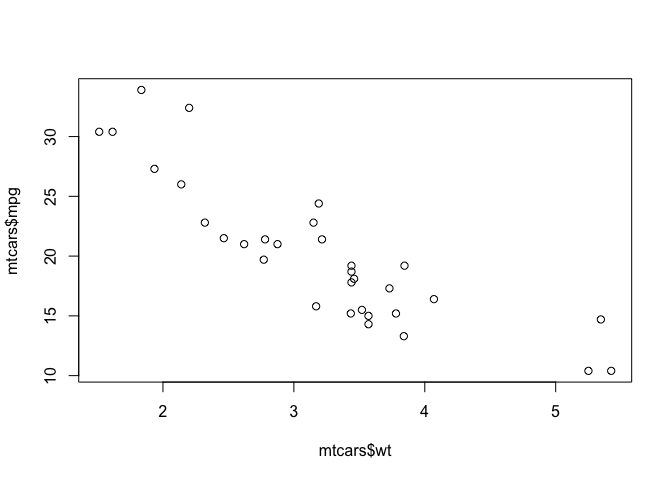Or you can use the more common formula notation:

``plot(mpg~wt,data=mtcars)``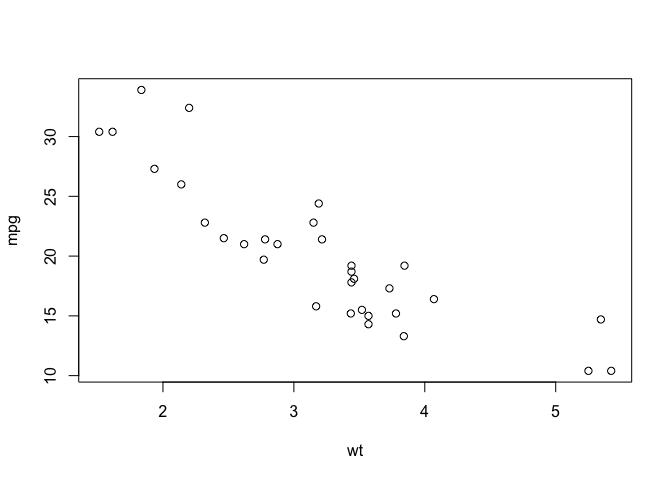And you can customize with various parameters:

``````plot(mpg~wt,data=mtcars,
ylab="Miles per gallon (mpg)",
xlab="Weight (1000 pounds)",
main="Fuel Efficiency vs. Weight",
col="red"
)``````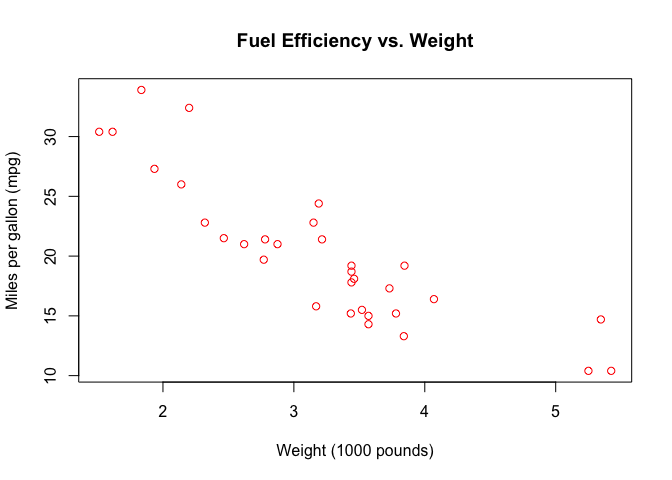Or switch to a line plot:

``````plot(mpg~wt,data=mtcars,
type="l",
ylab="Miles per gallon (mpg)",
xlab="Weight (1000 pounds)",
main="Fuel Efficiency vs. Weight",
col="blue"
)``````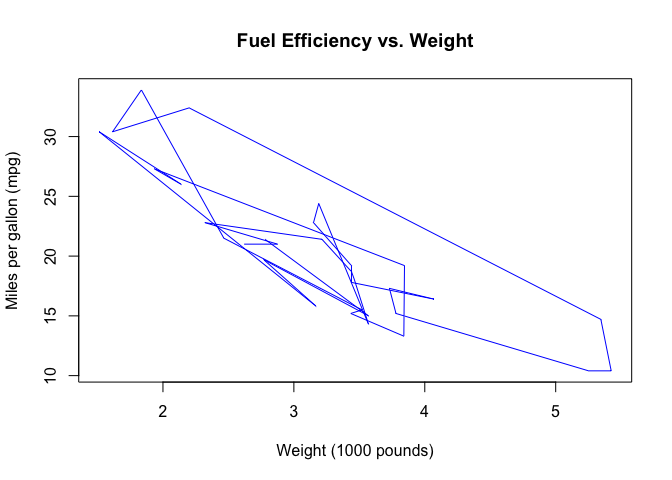See `?plot` for details.

## 1.2 Histograms

Check out the help for basic histograms.

``?hist``

Plot a histogram of the fuel efficiencies in the `mtcars` dataset.

``hist(mtcars\$mpg)``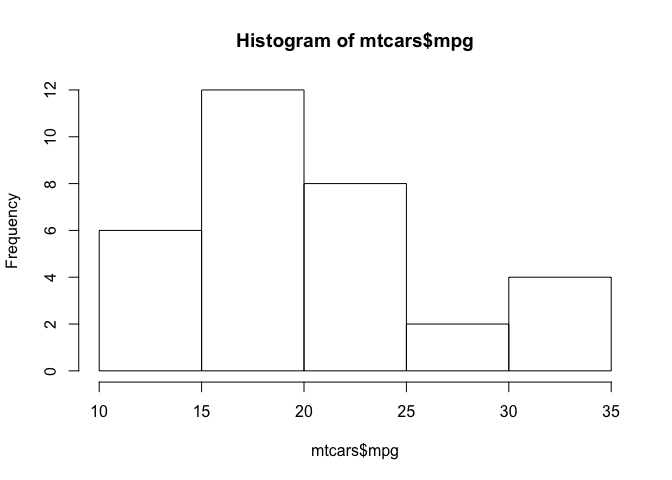# 2`ggplot2`

The grammar of graphics: consistent aesthetics, multidimensional conditioning, and step-by-step plot building.

1. Data: The raw data
2. `geom_`: The geometric shapes representing data
3. `aes()`: Aesthetics of the geometric and statistical objects (color, size, shape, and position)
4. `scale_`: Maps between the data and the aesthetic dimensions
``````data
+ geometry,
+ aesthetic mappings like position, color and size
+ scaling of ranges of the data to ranges of the aesthetics``````

1. `stat_`: Statistical summaries of the data that can be plotted, such as quantiles, fitted curves (loess, linear models), etc.
2. `coord_`: Transformation for mapping data coordinates into the plane of the data rectangle
3. `facet_`: Arrangement of data into grid of plots
4. `theme`: Visual defaults (background, grids, axes, typeface, colors, etc.)

For example, a simple scatterplot: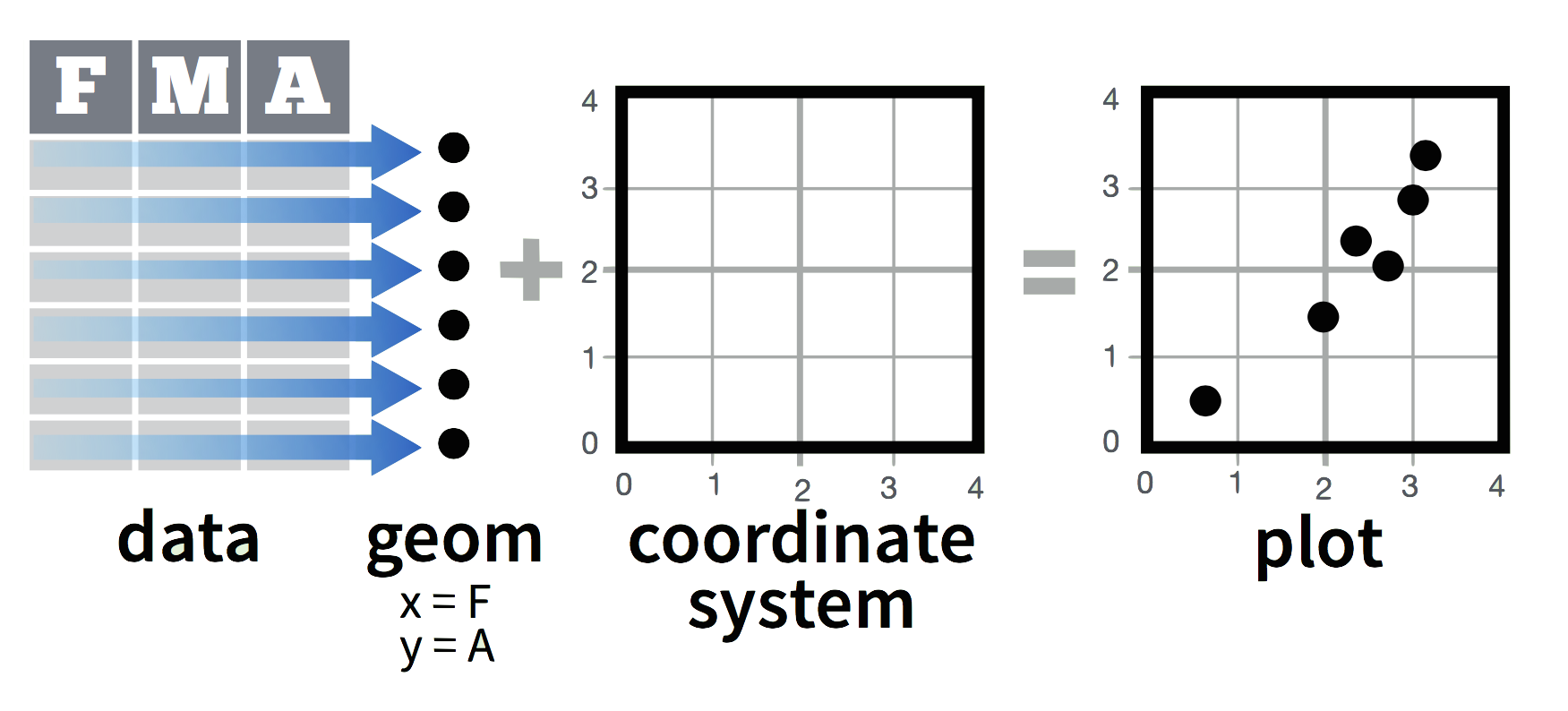Add variable colors and sizes: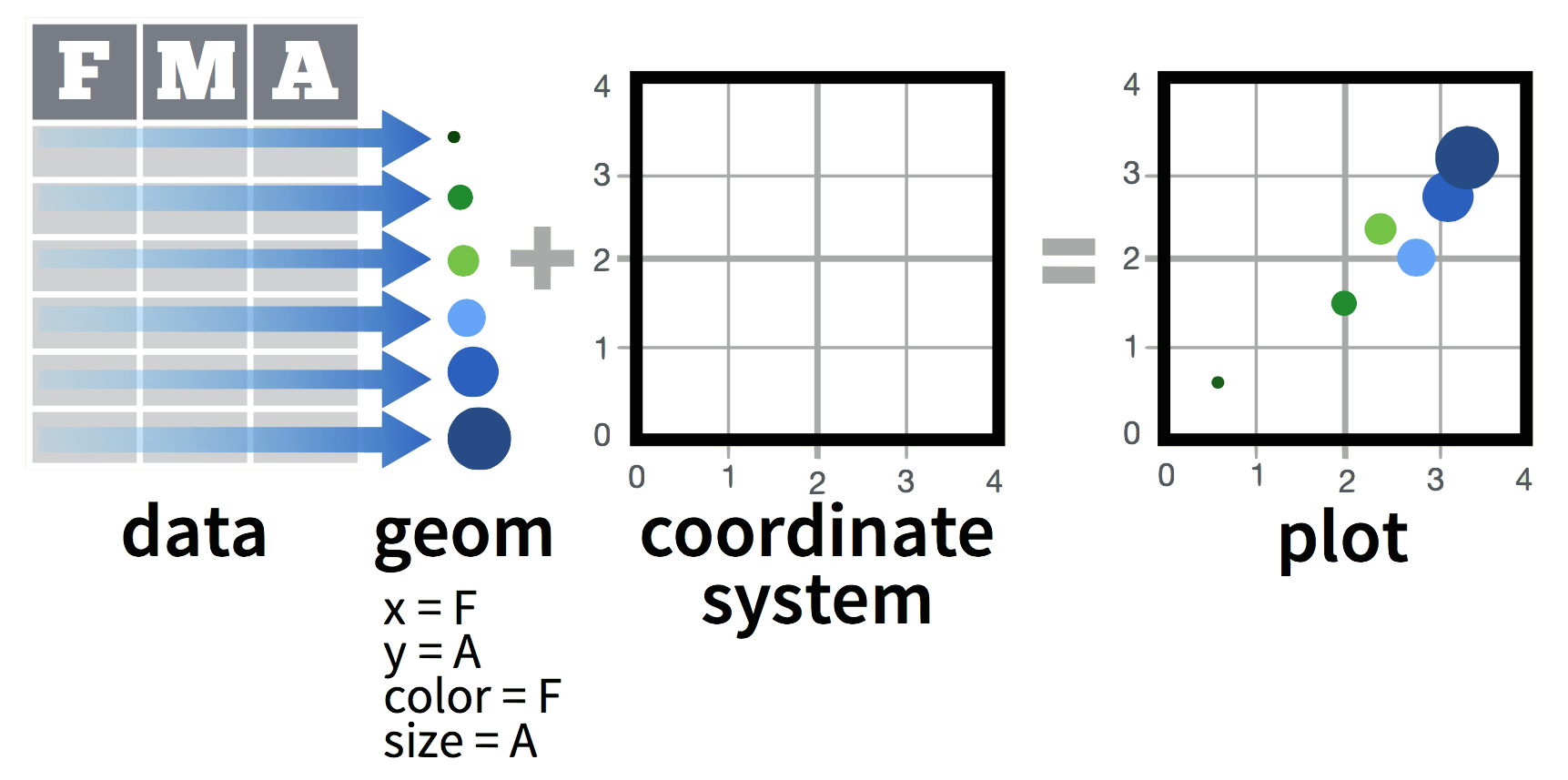## 2.1 Simple scatterplot

First, create a blank ggplot object with the data and x-y geometry set up.

``````p <- ggplot(mtcars, aes(x=wt, y=mpg))
summary(p)``````
``````## data: mpg, cyl, disp, hp, drat, wt, qsec,
##   vs, am, gear, carb [32x11]
## mapping:  x = wt, y = mpg
## faceting: <ggproto object: Class FacetNull, Facet>
##     compute_layout: function
##     draw_back: function
##     draw_front: function
##     draw_labels: function
##     draw_panels: function
##     finish_data: function
##     init_scales: function
##     map: function
##     map_data: function
##     params: list
##     render_back: function
##     render_front: function
##     render_panels: function
##     setup_data: function
##     setup_params: function
##     shrink: TRUE
##     train: function
##     train_positions: function
##     train_scales: function
##     vars: function
##     super:  <ggproto object: Class FacetNull, Facet>``````
``p``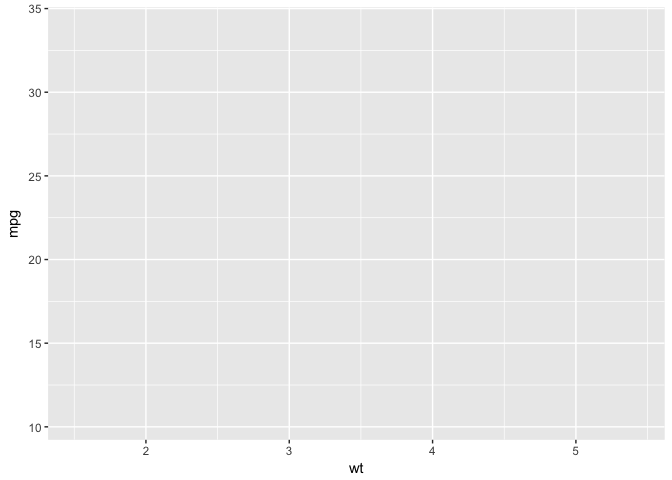``p + geom_point()``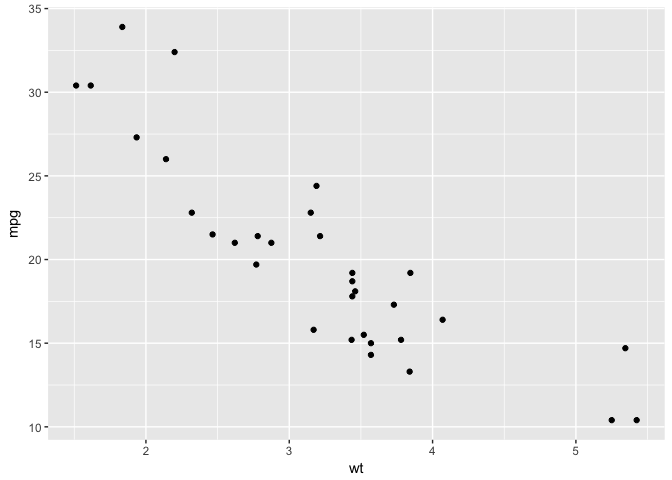Or you can do both at the same time:

``````ggplot(mtcars, aes(x=wt, y=mpg)) +
geom_point()``````### 2.1.1 Aesthetic map: color by # of cylinders

``````p +
geom_point(aes(colour = factor(cyl)))``````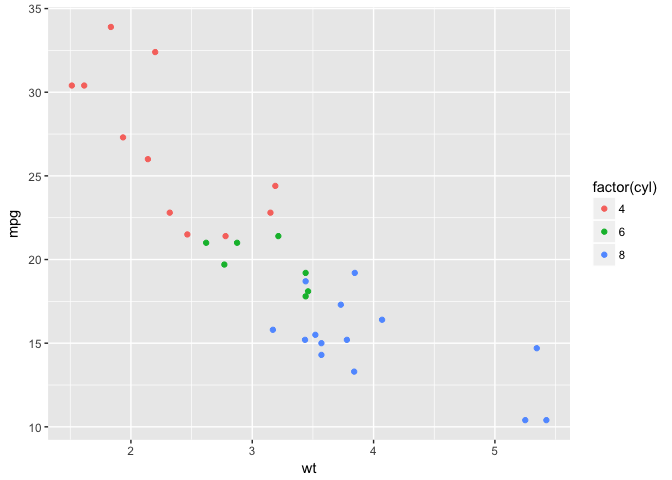### 2.1.2 Set shape using # of cylinders

``````p +
geom_point(aes(shape = factor(cyl)))``````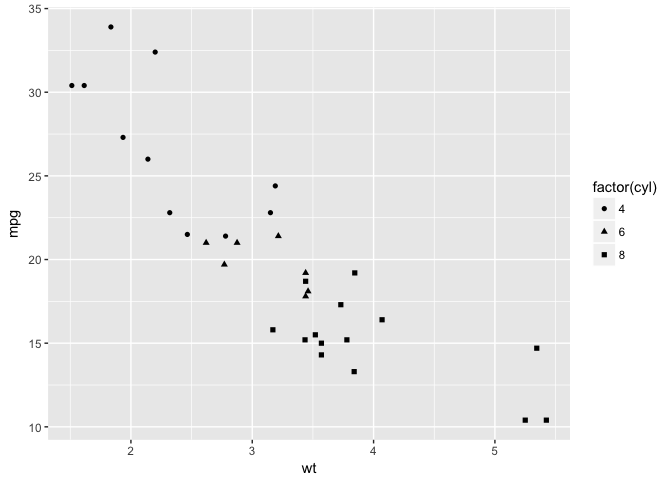### 2.1.3 Adjust size by `qsec`

``````p +
geom_point(aes(size = qsec))``````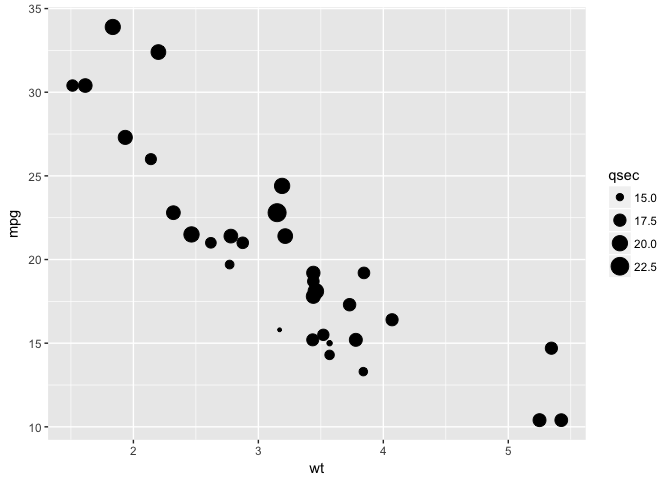### 2.1.4 Color by cylinders and size by `qsec`

``````p +
geom_point(aes(colour = factor(cyl),size = qsec))``````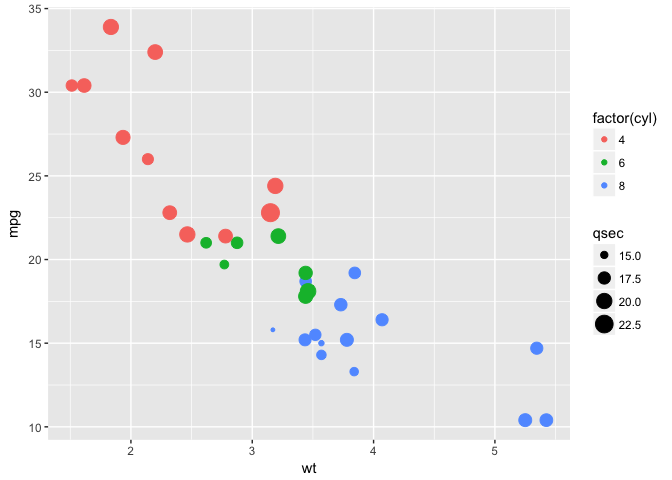### 2.1.5 Multiple aesthetics

``````p +
geom_point(aes(colour = factor(cyl),size = qsec,shape=factor(gear)))``````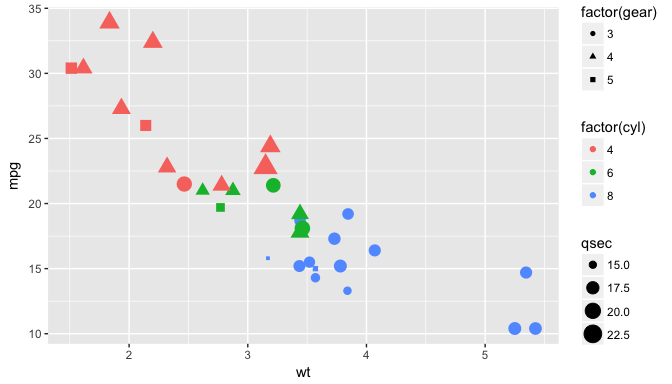### 2.1.6 Add a linear model

``````p + geom_point() +
geom_smooth(method="lm")``````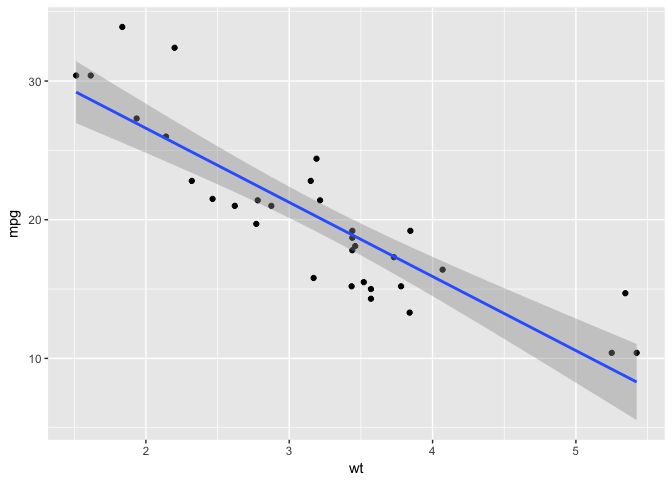### 2.1.7 Add a LOESS smooth

``````p + geom_point() +
geom_smooth(method="loess")``````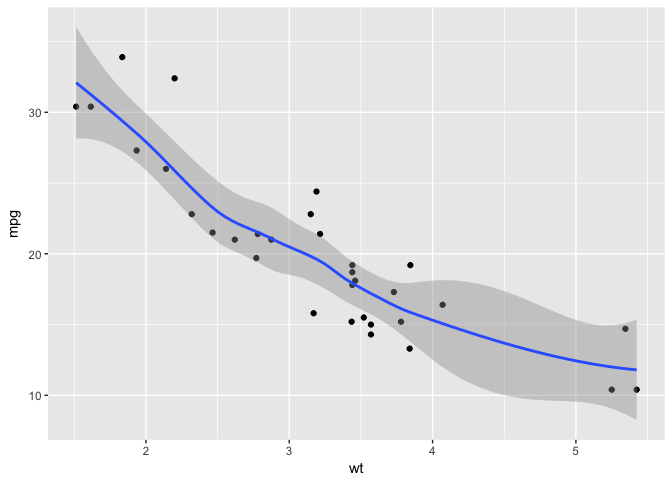### 2.1.8 Change scale color

``````p + geom_point(aes(colour = cyl)) +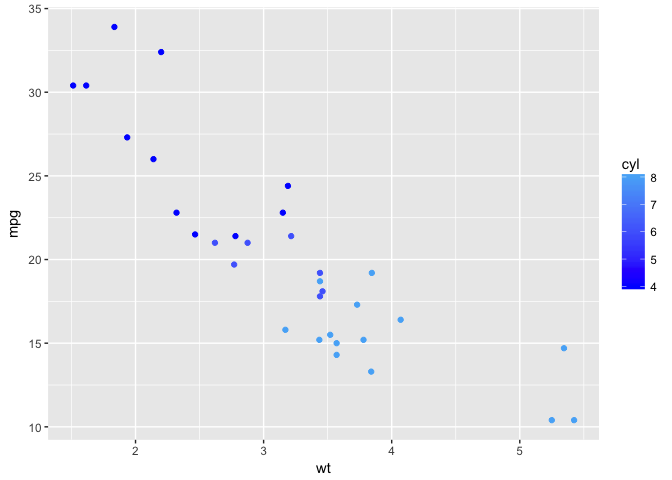### 2.1.9 Change scale shapes

``````p + geom_point(aes(shape = factor(cyl))) +
scale_shape(solid = FALSE)``````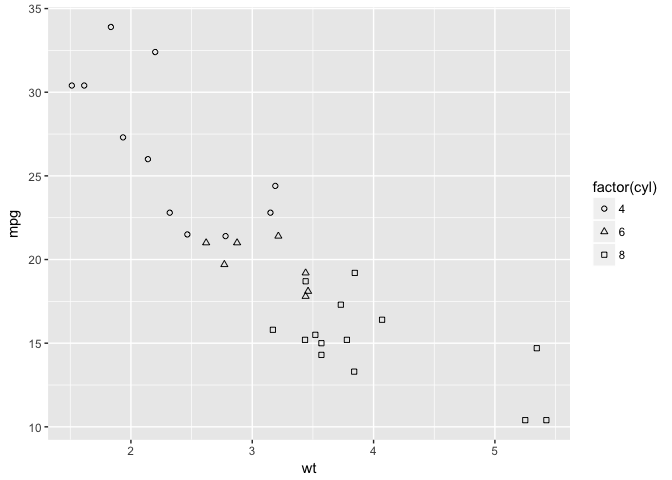### 2.1.10 Set aesthetics to fixed value

``````ggplot(mtcars, aes(wt, mpg)) +
geom_point(colour = "red", size = 3)``````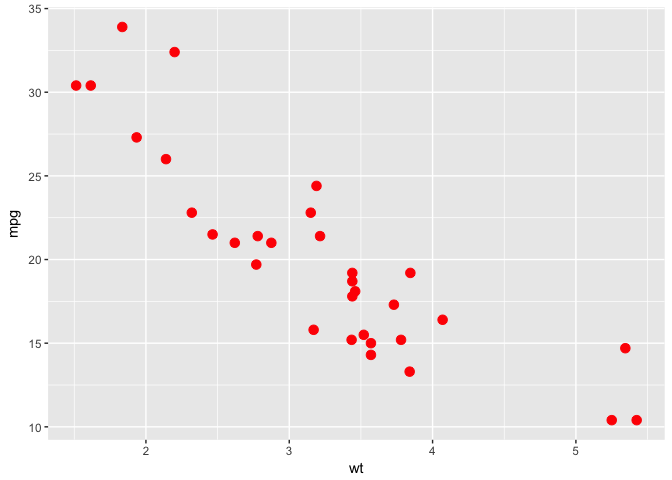### 2.1.11 Transparancy: alpha=0.2

``````d <- ggplot(diamonds, aes(carat, price))
d + geom_point(alpha = 0.2)``````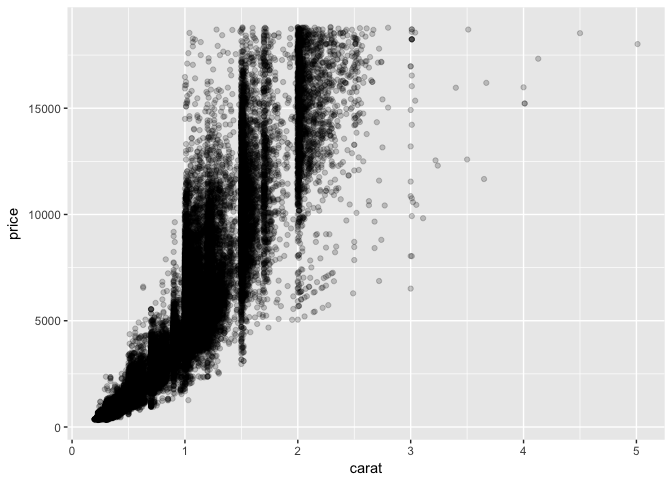Varying alpha useful for large data sets

### 2.1.12 Transparancy: alpha=0.1

``````d +
geom_point(alpha = 0.1)``````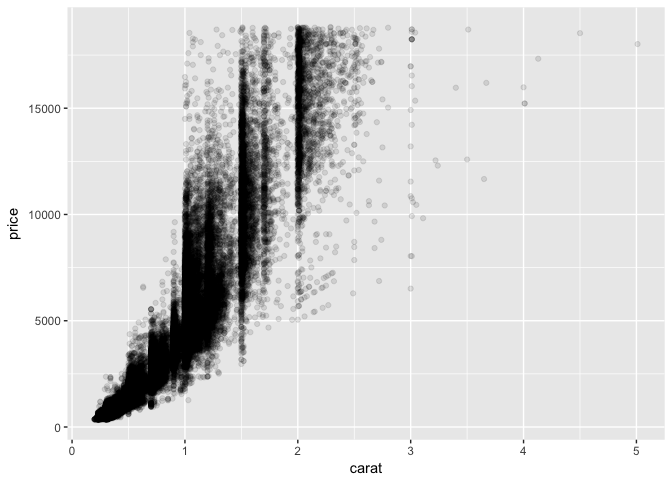### 2.1.13 Transparancy: alpha=0.01

``````d +
geom_point(alpha = 0.01)``````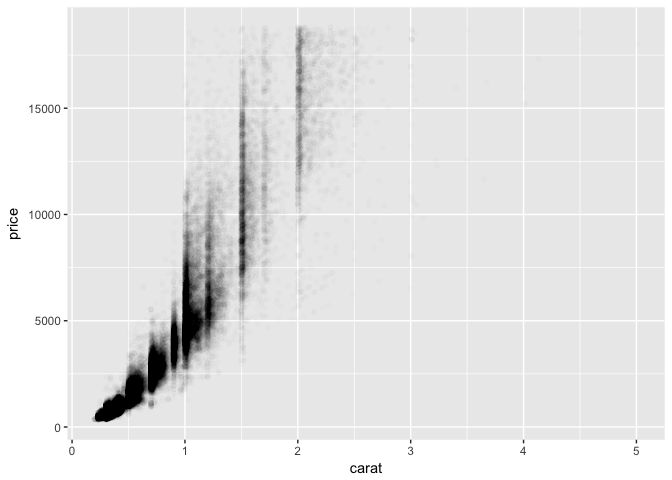## 2.2 Building ggplots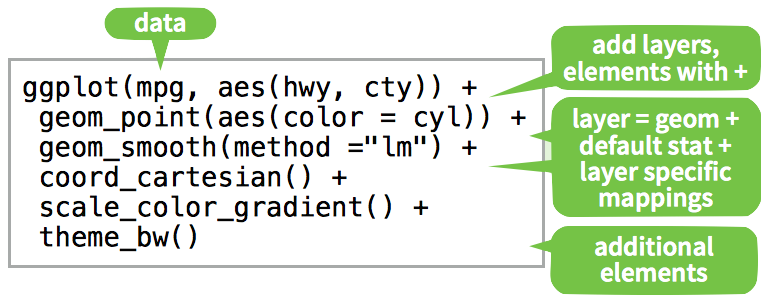## 2.3 Other Plot types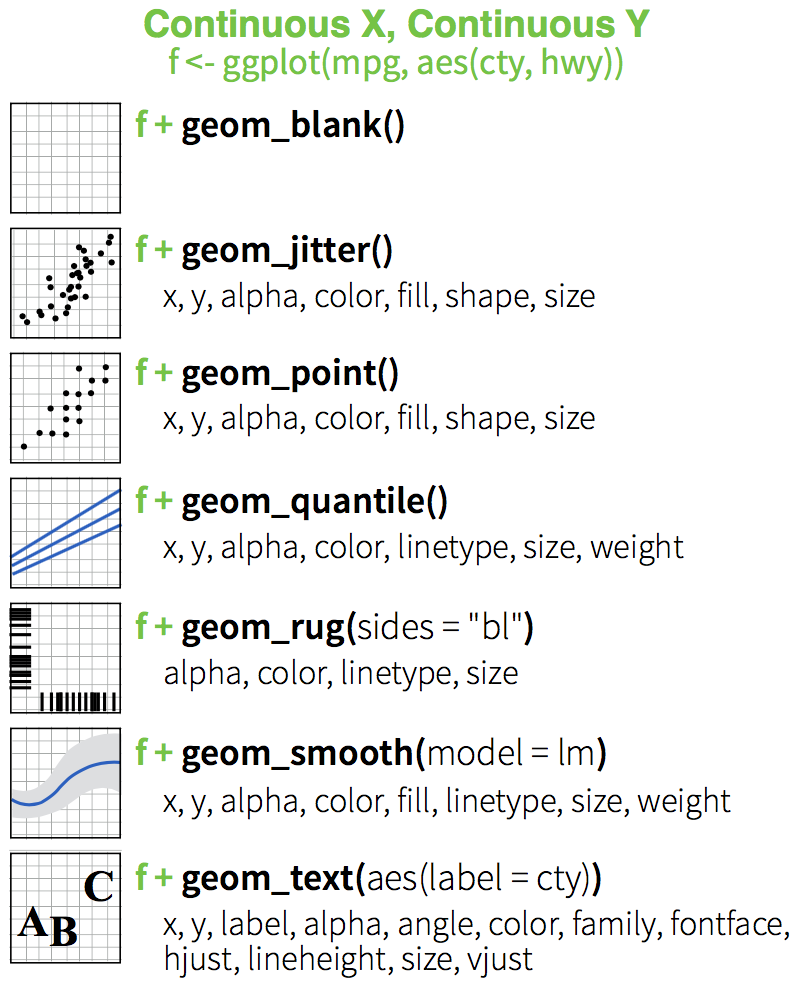Edit plot `p` to include:

1. points
2. A smooth (‘loess’) curve
3. a “rug” to the plot
``p <- ggplot(mtcars, aes(x=wt, y=mpg))``
``````p+
geom_point()+
geom_smooth()+
geom_rug()``````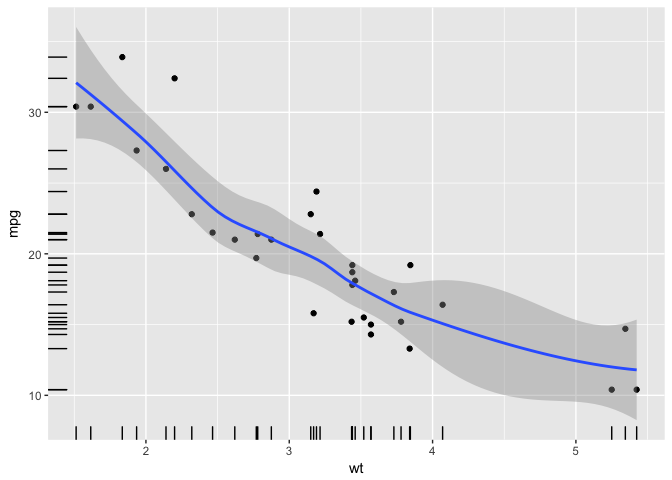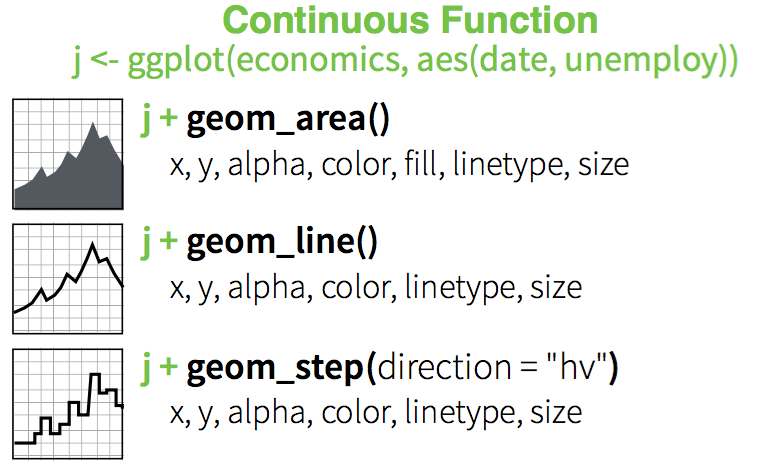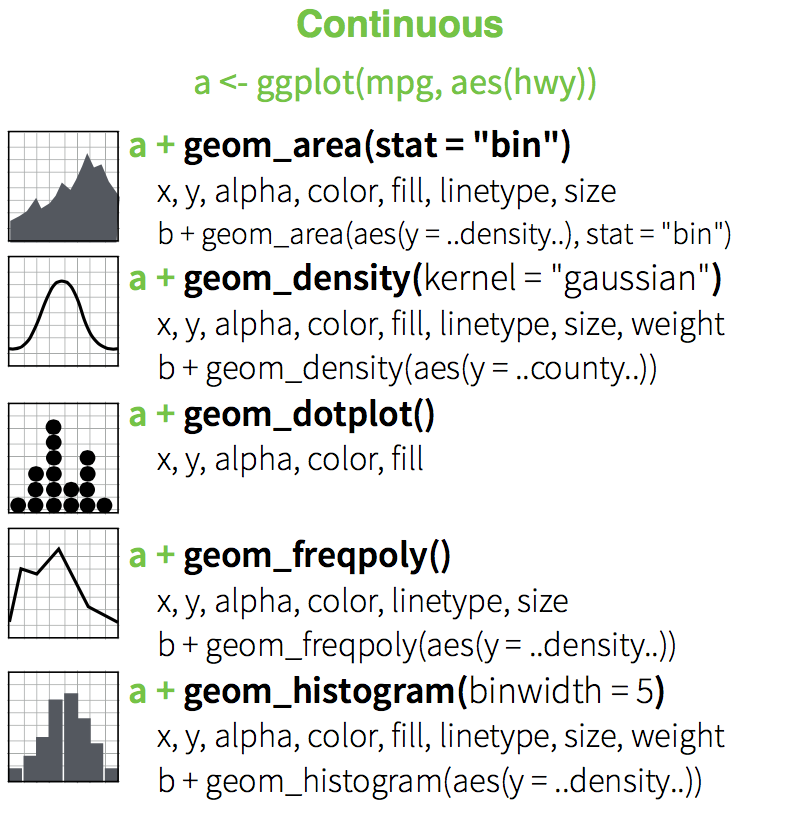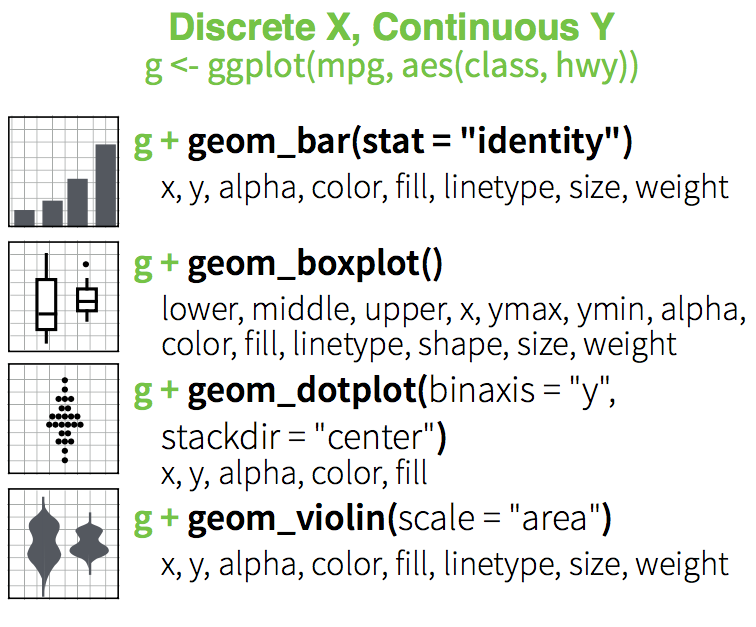### 2.3.1 Discrete X, Continuous Y

``````p <- ggplot(mtcars, aes(factor(cyl), mpg))
p + geom_point()``````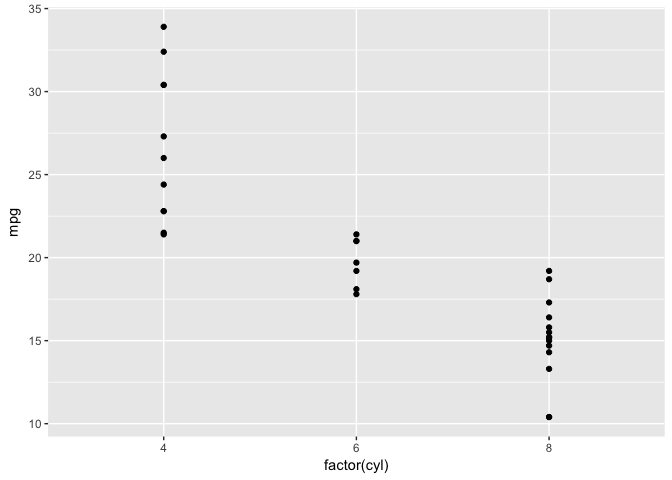### 2.3.2 Discrete X, Continuous Y + geom_jitter()

``````p +
geom_jitter()``````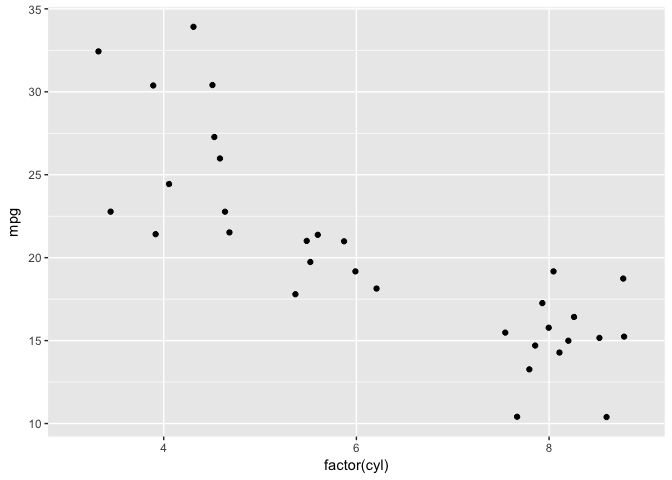### 2.3.3 Discrete X, Continuous Y + geom_violin()

``````p +
geom_violin()``````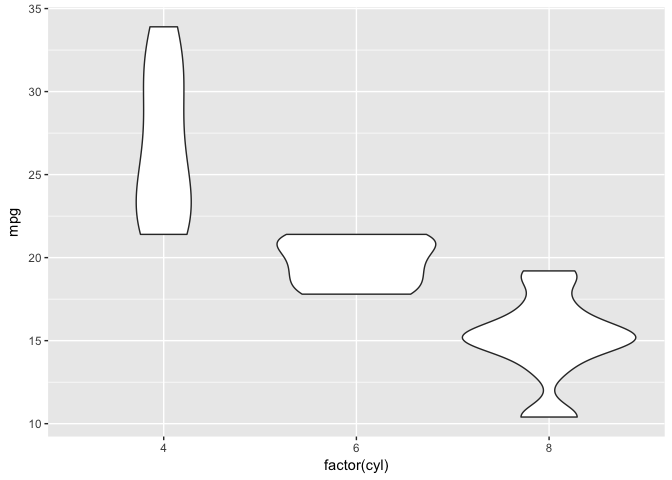### 2.3.4 Discrete X, Continuous Y + geom_violin()

``````p +
geom_violin() + geom_jitter(position = position_jitter(width = .1))``````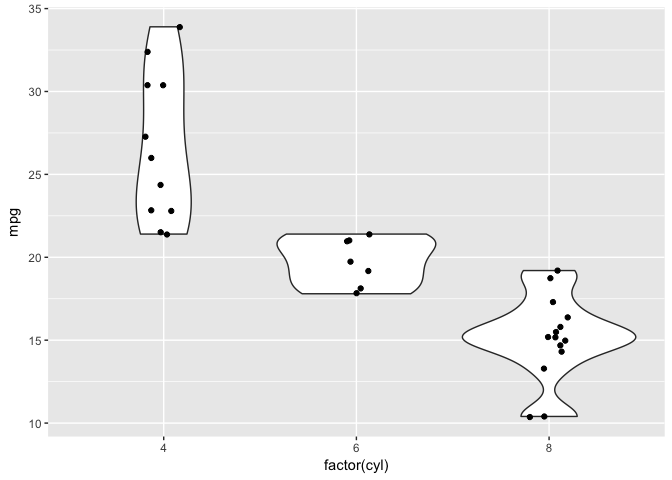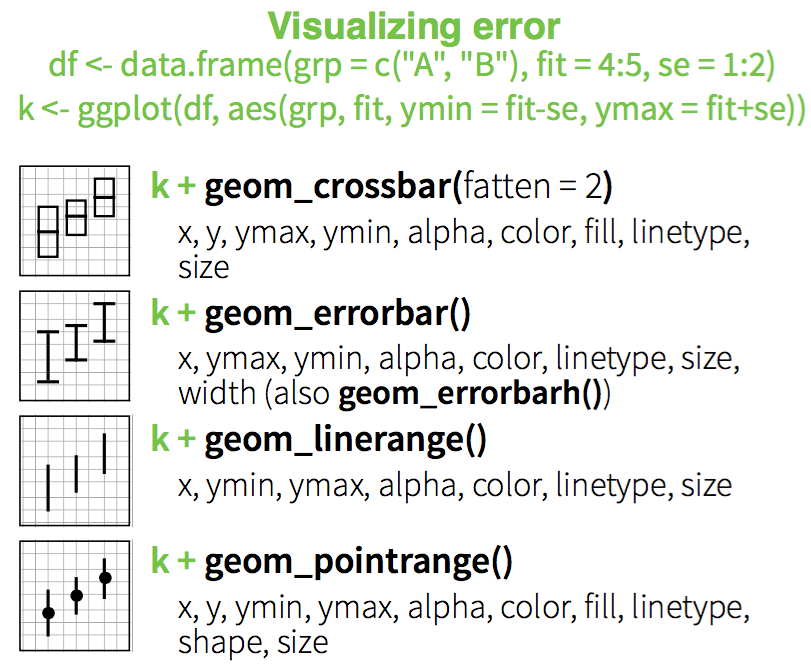### 2.3.5 Three Variables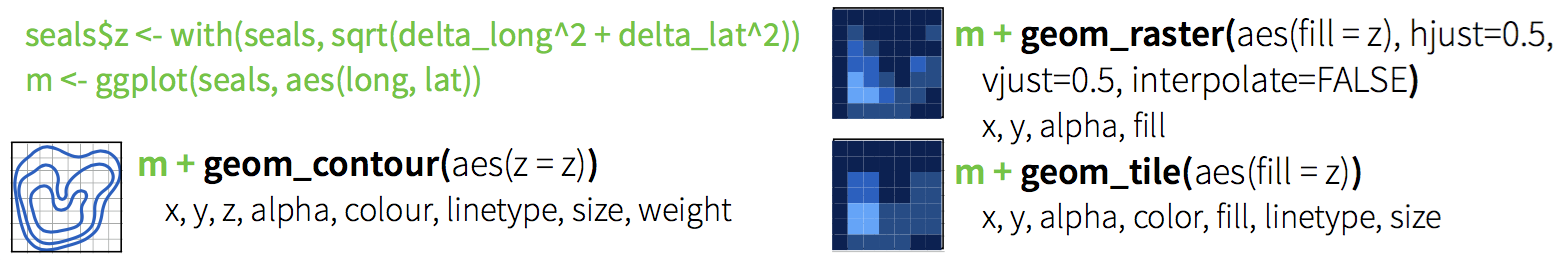### 2.3.6 Stats

Visualize a data transformation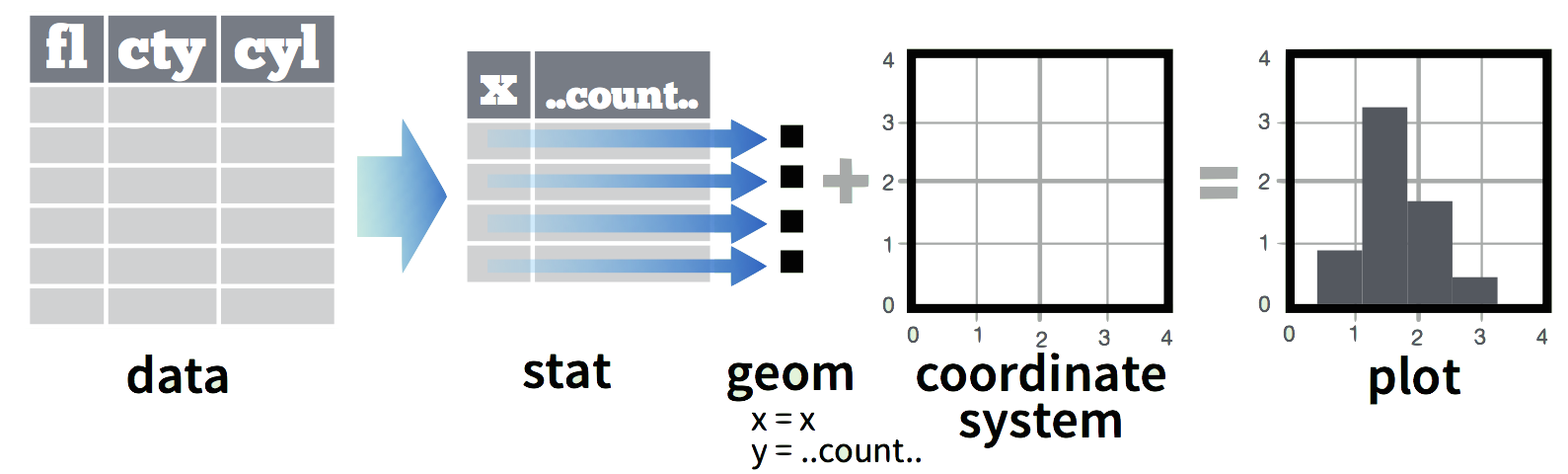• Each stat creates additional variables with a common `..name..` syntax
• Often two ways: `stat_bin(geom="bar")` OR `geom_bar(stat="bin")`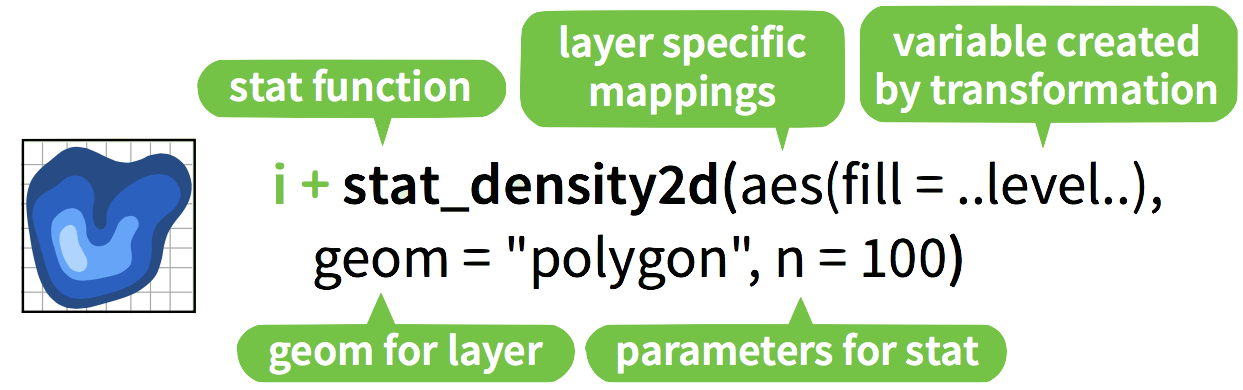### 2.3.7 2D kernel density estimation

Old Faithful Geyser Data on duration and waiting times.

``library("MASS")``
``````##
## Attaching package: 'MASS'``````
``````## The following object is masked from 'package:dplyr':
##
##     select``````
``````## The following objects are masked from 'package:raster':
##
##     area, select``````
``````data(geyser)
m <- ggplot(geyser, aes(x = duration, y = waiting))``````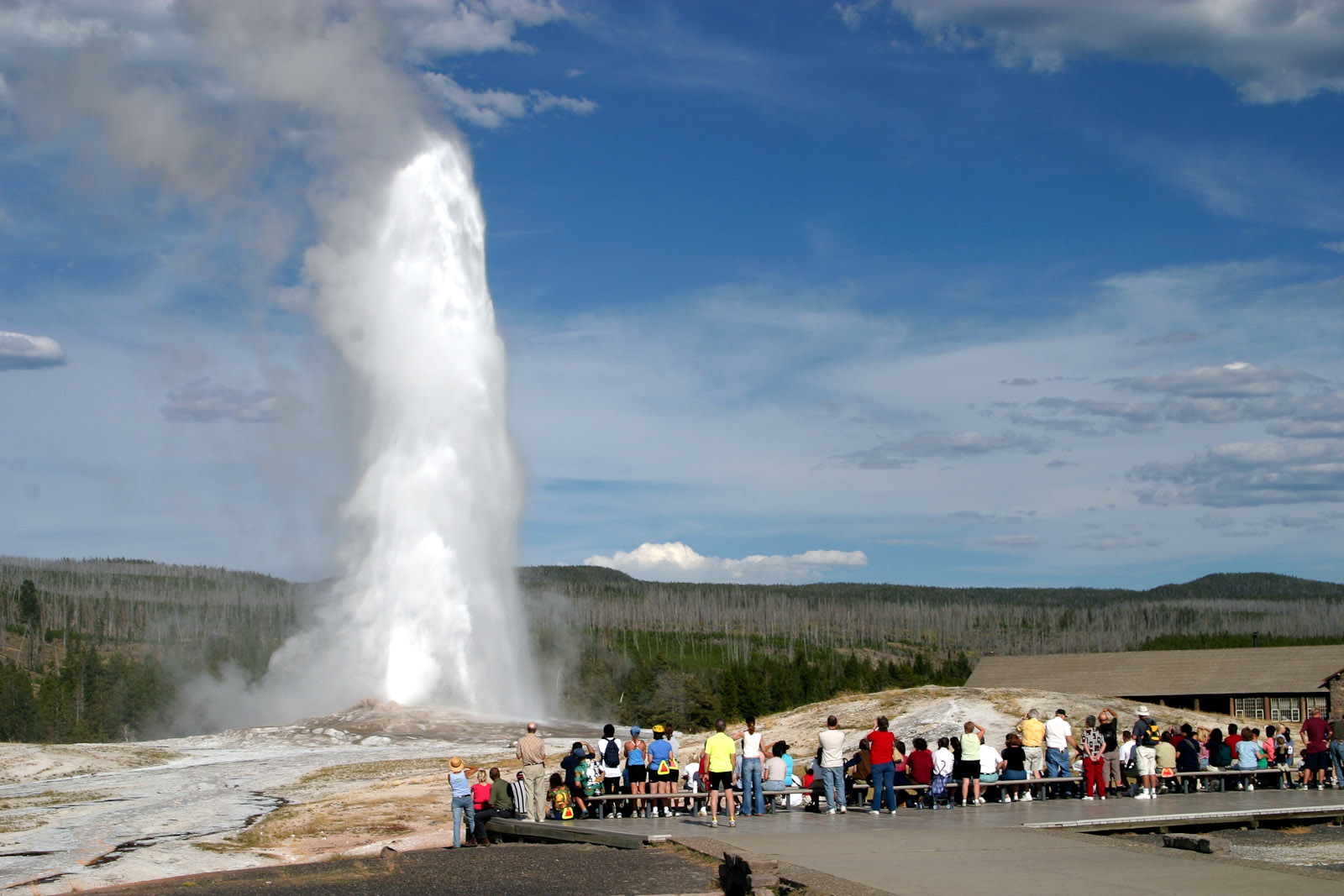photo: Greg Willis

See `?geyser` for details.

``````m +
geom_point()``````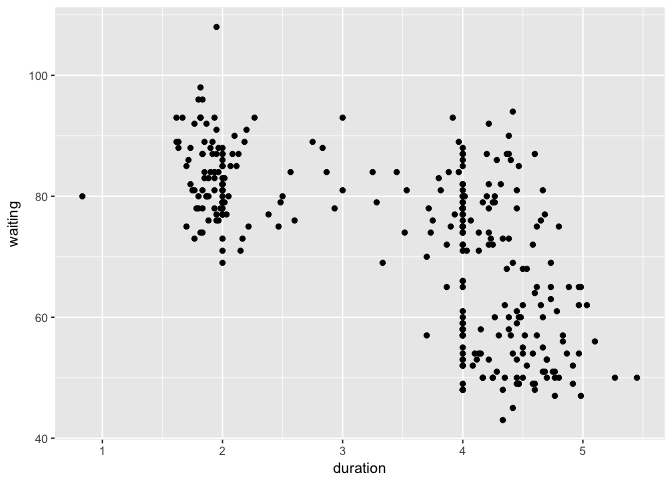``````m +
geom_point() +  stat_density2d(geom="contour")``````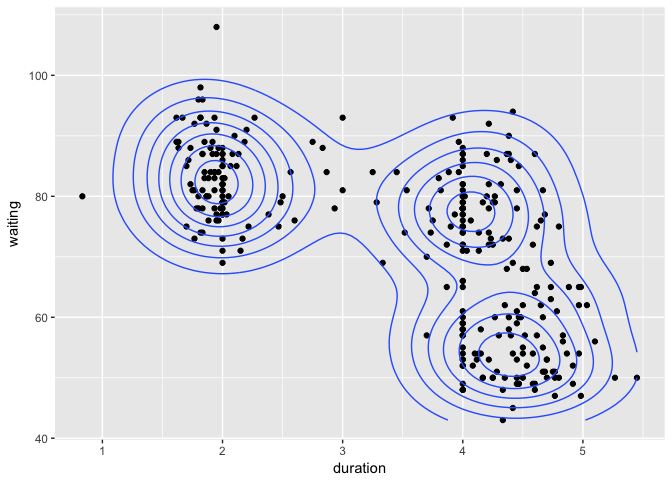Check `?geom_density2d()` for details

``````m +
geom_point() +  stat_density2d(geom="contour") +
xlim(0.5, 6) + ylim(40, 110)``````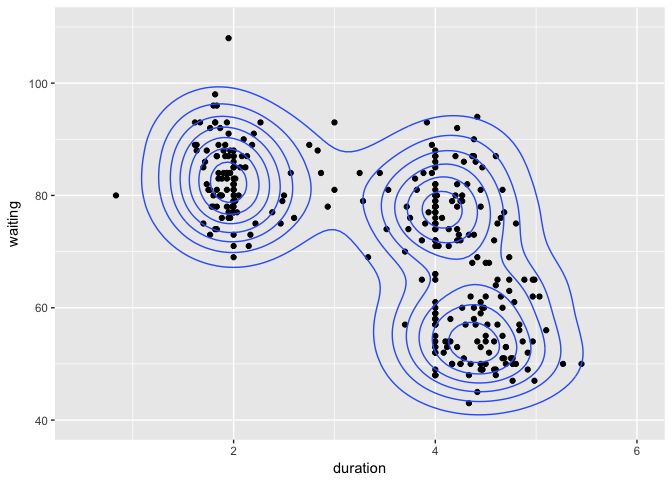Update limits to show full contours. Check `?geom_density2d()` for details

``````m + stat_density2d(aes(fill = ..level..), geom="polygon") +
geom_point(col="red")``````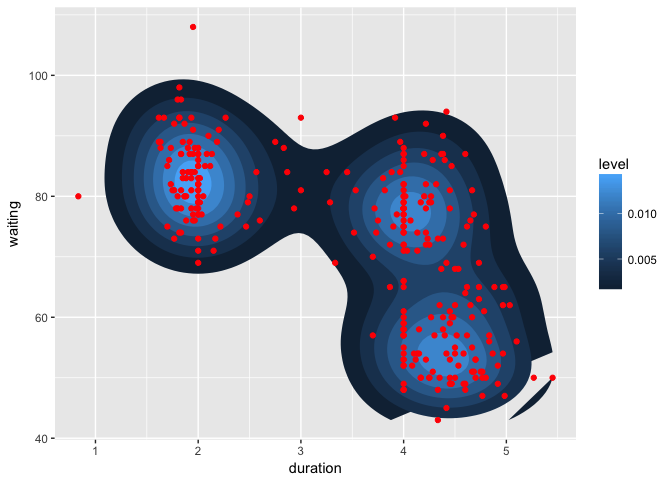Check `?geom_density2d()` for details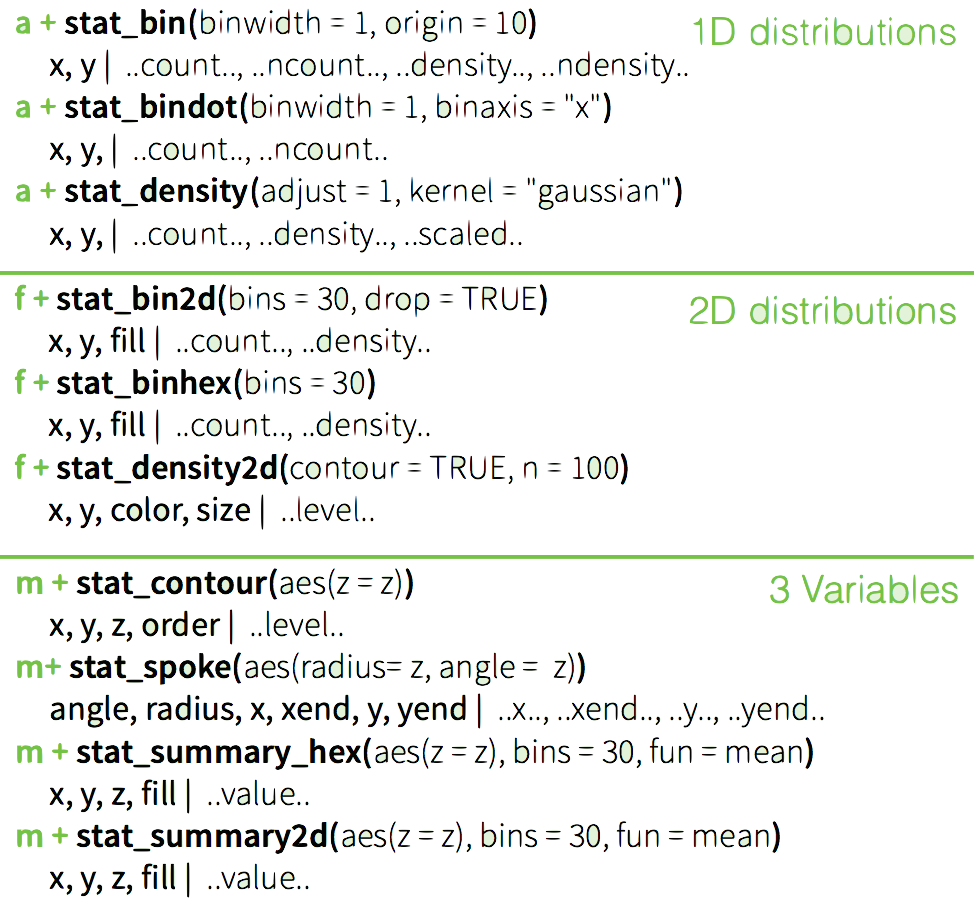Edit plot `m` to include:

• The point data (with red points) on top
• A `binhex` plot of the Old Faithful data

Experiment with the number of bins to find one that works.

See `?stat_binhex` for details.

``m <- ggplot(geyser, aes(x = duration, y = waiting))``
``````m + stat_binhex(bins=10) +
geom_point(col="red")``````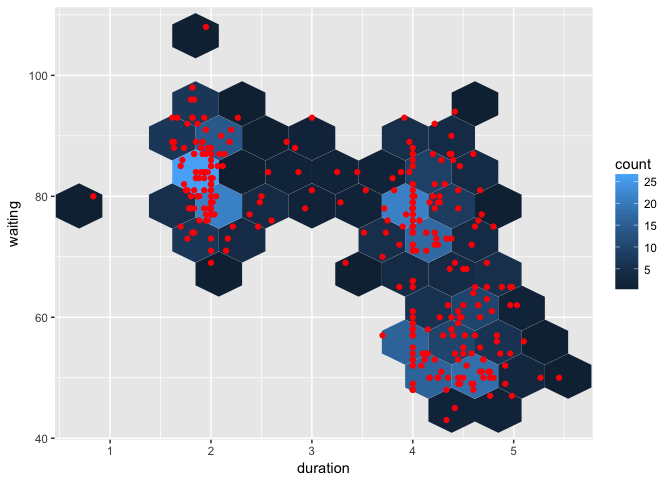## 2.4 Specifying Scales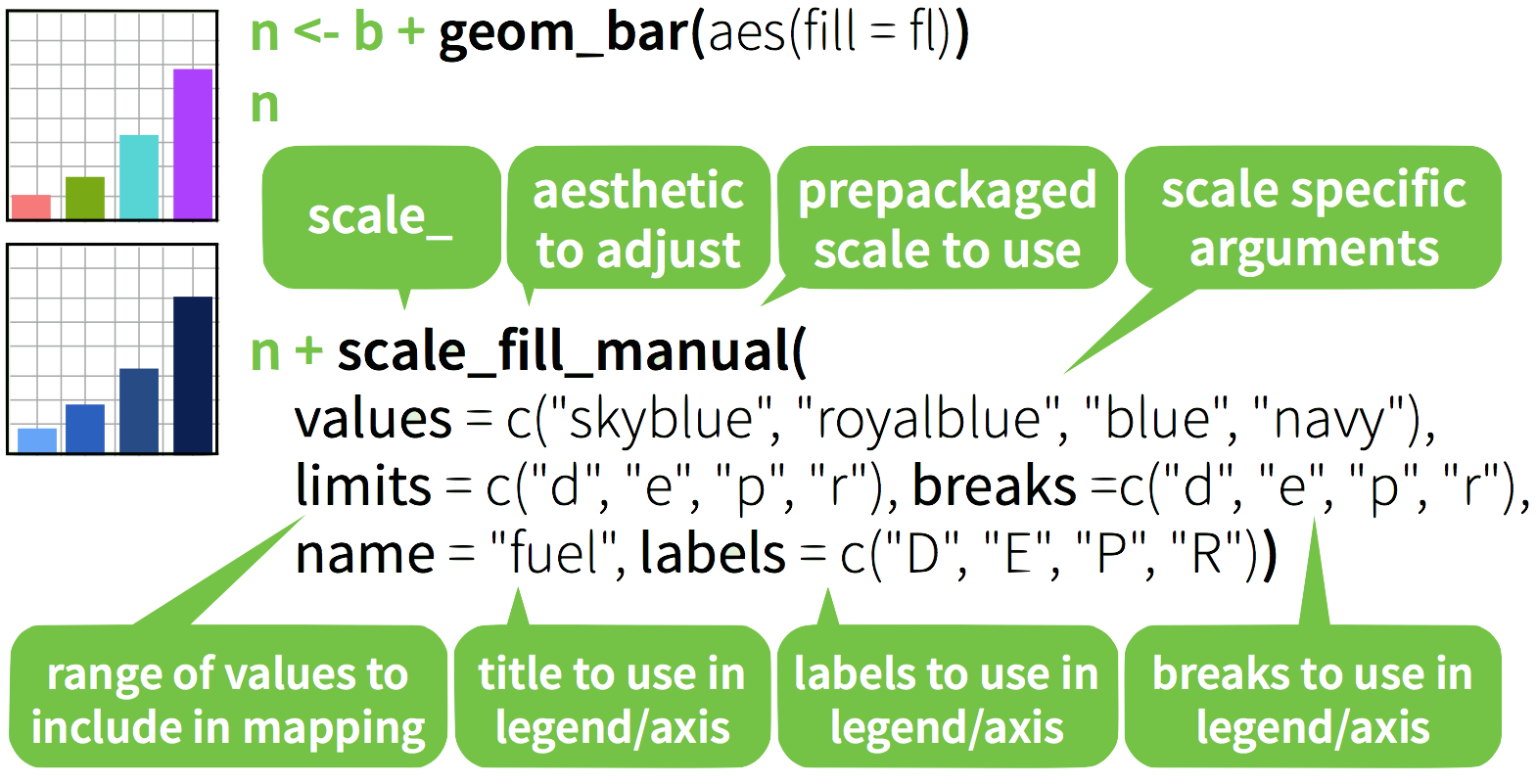### 2.4.1 Discrete color: default

``````b=ggplot(mpg,aes(fl))+
geom_bar( aes(fill = fl)); b``````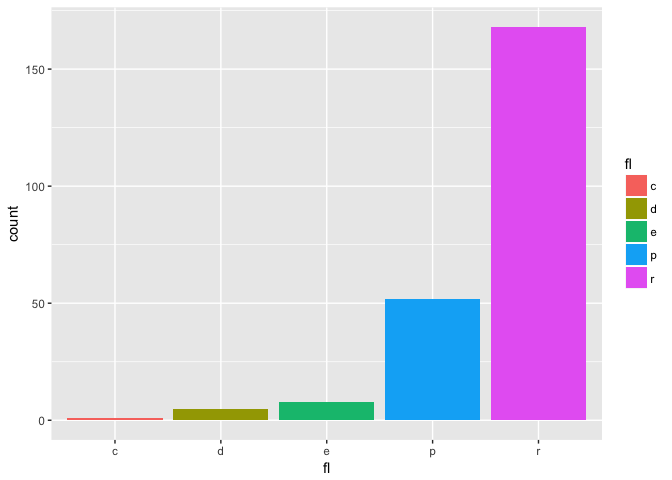### 2.4.2 Discrete color: greys

``````b + scale_fill_grey( start = 0.2, end = 0.8,
na.value = "red")``````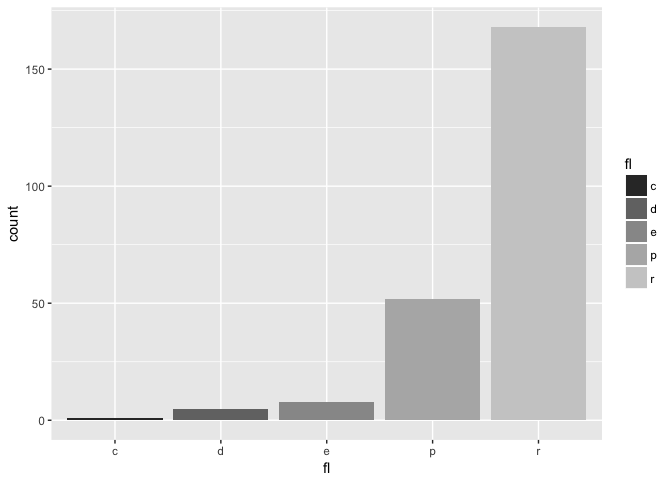### 2.4.3 Continuous color: defaults

``````a <- ggplot(mpg, aes(x=hwy,y=cty,col=displ)) +
geom_point(); a``````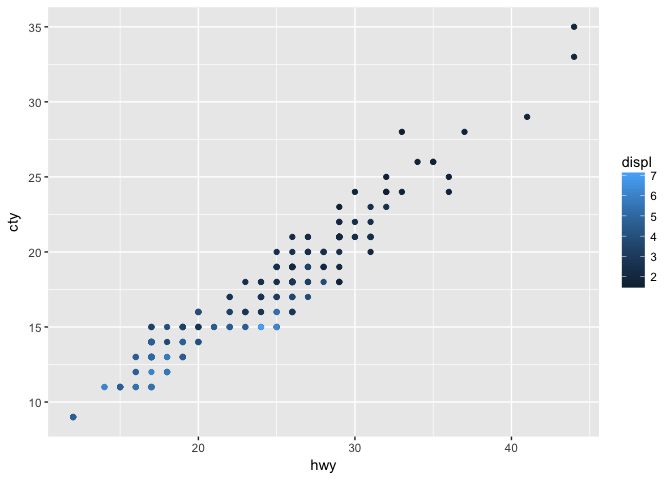### 2.4.4 Continuous color: `gradient`

``````a +  scale_color_gradient( low = "red",
high = "yellow")``````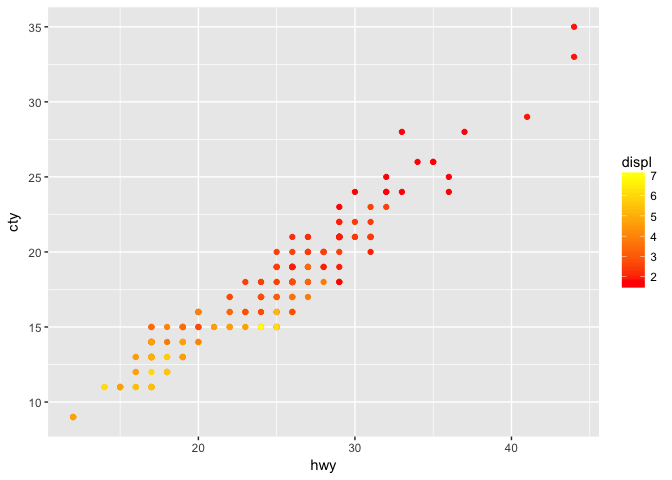### 2.4.5 Continuous color: `gradient2`

``````a + scale_color_gradient2(low = "red", high = "blue",
mid = "white", midpoint = 4)``````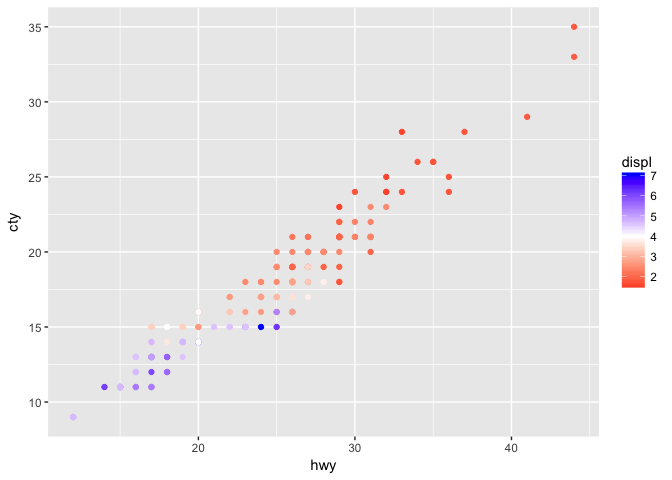### 2.4.6 Continuous color: `gradientn`

``````a + scale_color_gradientn(
colours = rainbow(10))``````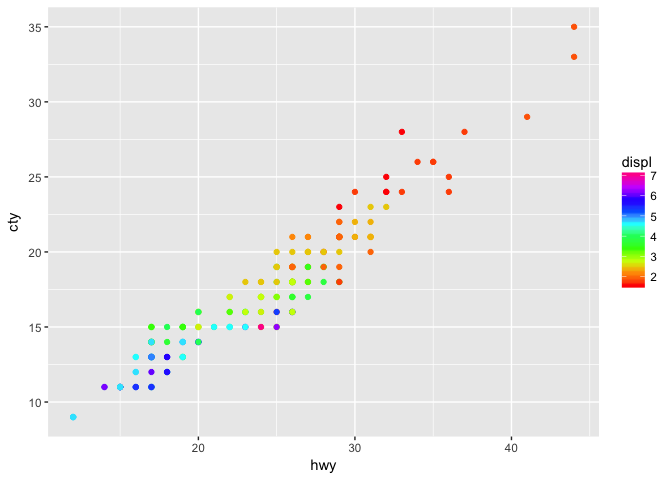### 2.4.7 Discrete color: brewer

``````b +
scale_fill_brewer( palette = "Blues")``````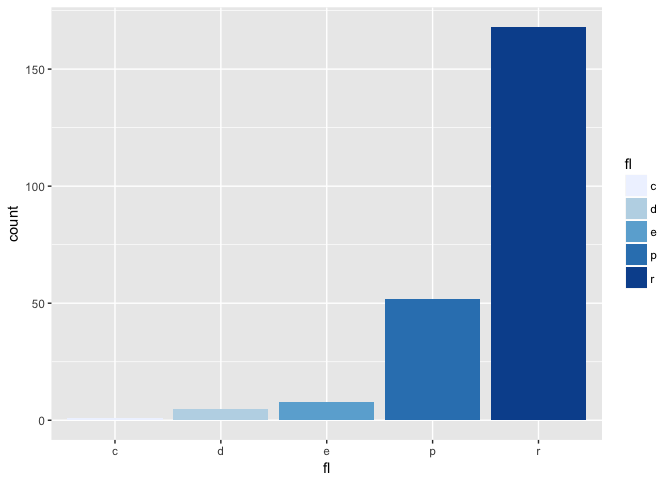## 2.5colorbrewer2.org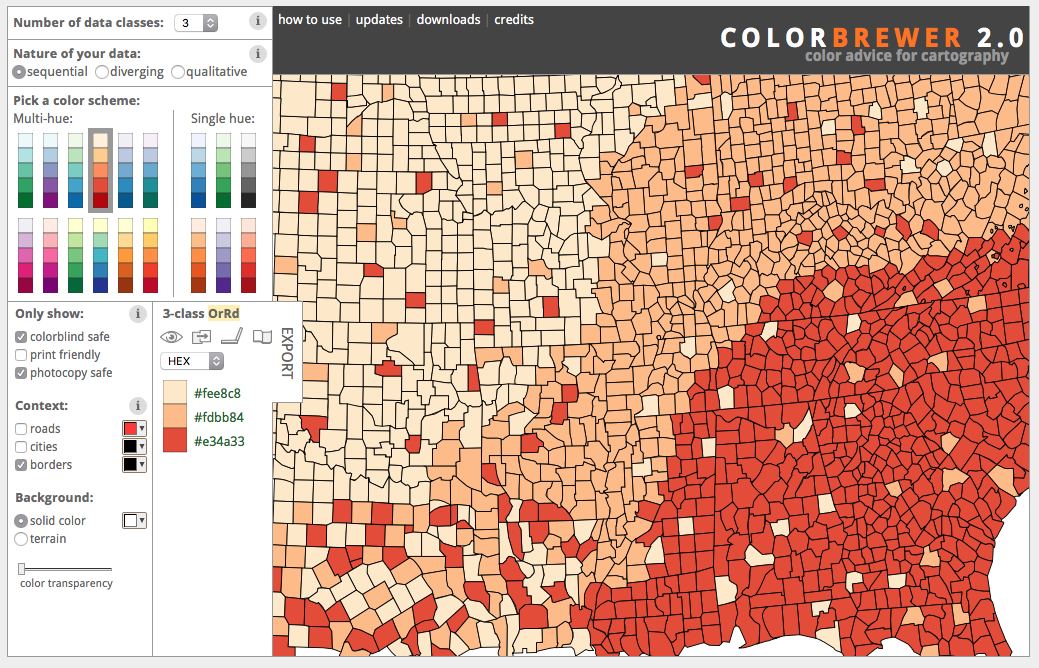## 2.6 ColorBrewer: Diverging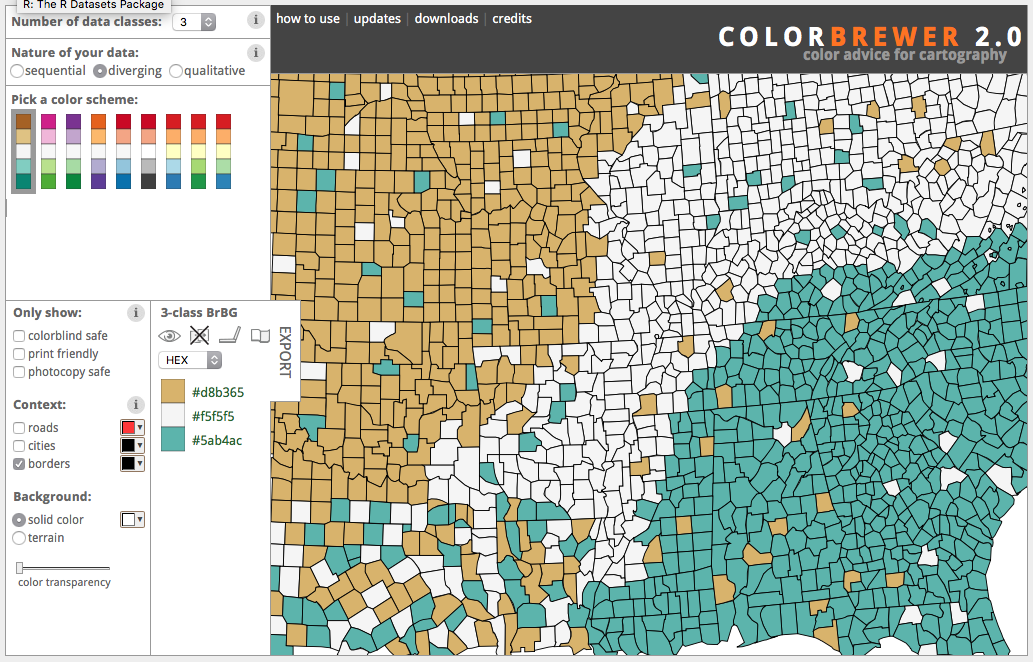## 2.7 ColorBrewer: Filtered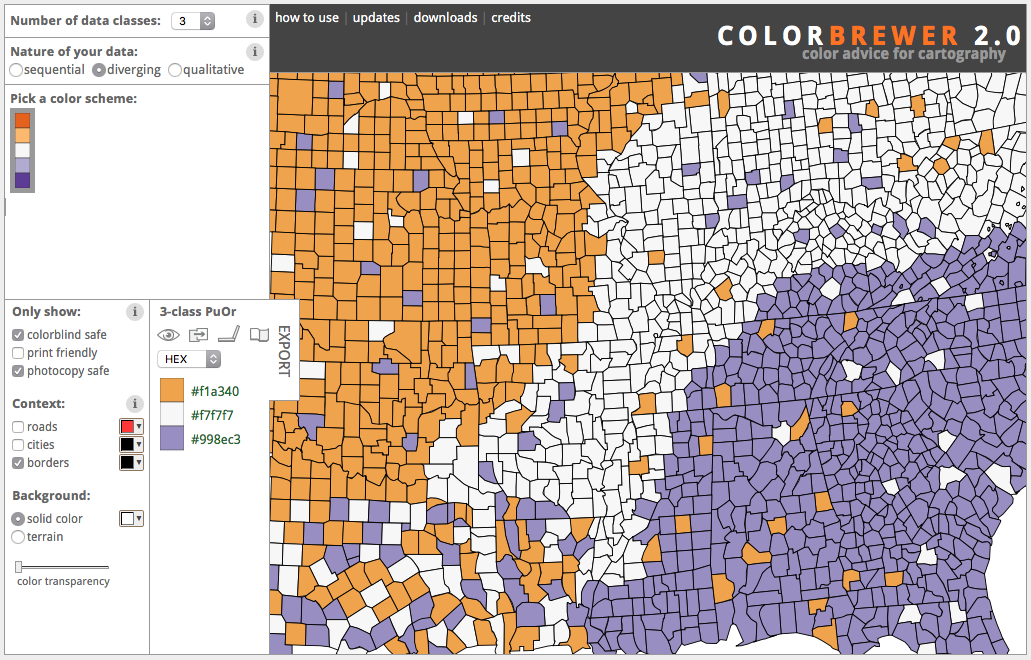Edit the contour plot of the geyser data:

1. Reduce the size of the points
2. Use a sequential brewer palette (select from colorbrewer2.org)
3. Add informative x and y labels
``````m <- ggplot(geyser, aes(x = duration, y = waiting)) +
stat_density2d(aes(fill = ..level..), geom="polygon") +
geom_point(col="red")``````

Note: `scale_fill_distiller()` rather than `scale_fill_brewer()` for continuous data

``````m + stat_density2d(aes(fill = ..level..), geom="polygon") +
geom_point(size=.75)+
scale_fill_distiller(palette="OrRd",
name="Kernel\nDensity")+
xlim(0.5, 6) + ylim(40, 110)+
xlab("Eruption Duration (minutes)")+
ylab("Waiting time (minutes)")``````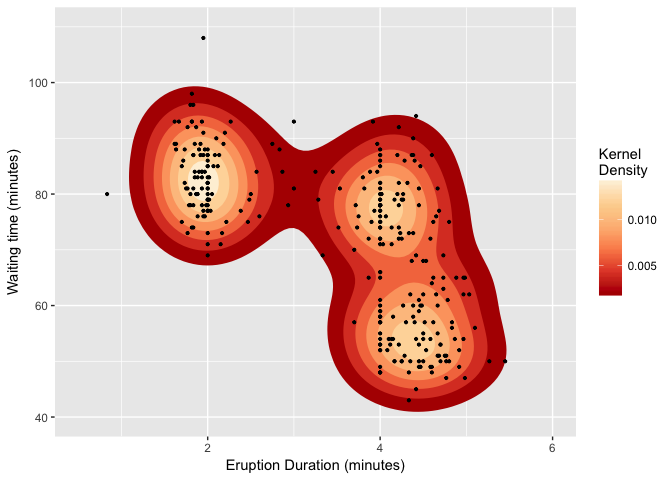Or use `geom=tile` for a raster representation.

``````m + stat_density2d(aes(fill = ..density..), geom="tile",contour=F) +
geom_point(size=.75)+
scale_fill_distiller(palette="OrRd",
name="Kernel\nDensity")+
xlim(0.5, 6) + ylim(40, 110)+
xlab("Eruption Duration (minutes)")+
ylab("Waiting time (minutes)")``````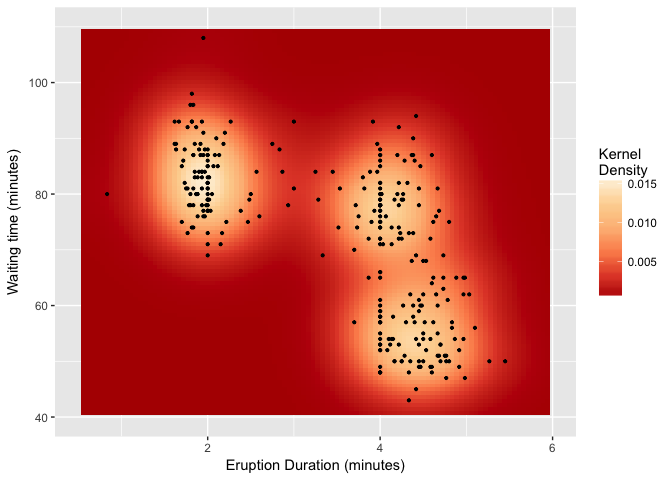## 2.8 Axis scaling

Create noisy exponential data

``````set.seed(201)
n <- 100
dat <- data.frame(
xval = (1:n+rnorm(n,sd=5))/20,
yval = 10^((1:n+rnorm(n,sd=5))/20)
)``````

Make scatter plot with regular (linear) axis scaling

``````sp <- ggplot(dat, aes(xval, yval)) + geom_point()
sp``````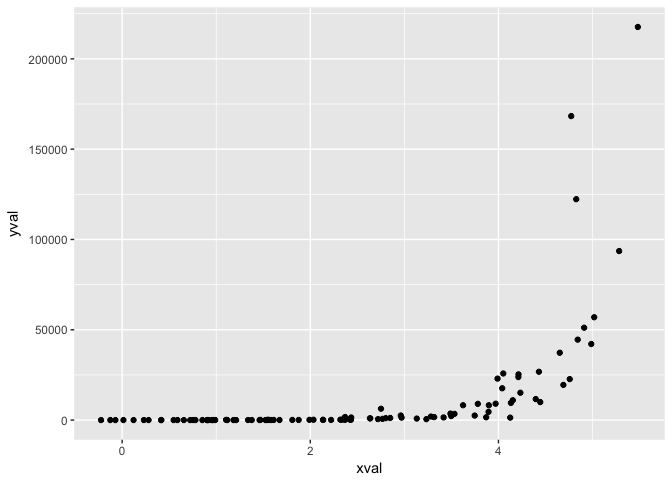Example from R Cookbook

log10 scaling of the y axis (with visually-equal spacing)

``sp + scale_y_log10()``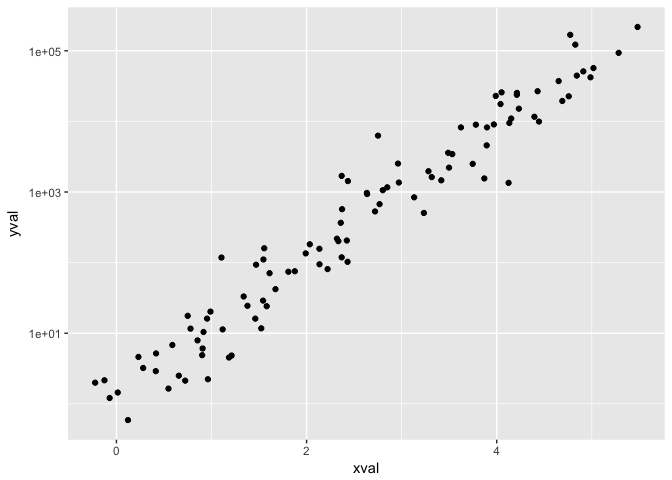## 2.9 Coordinate Systems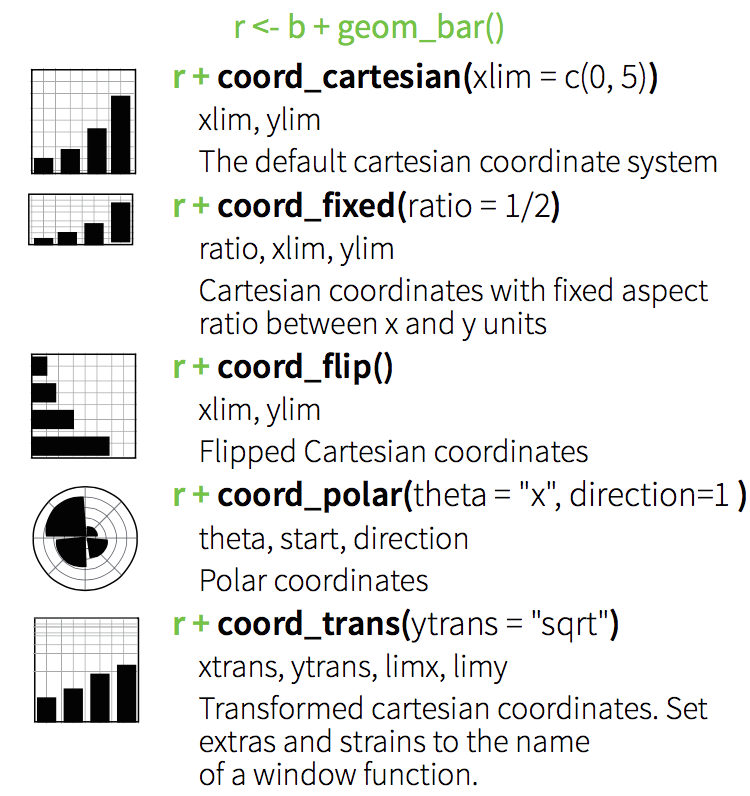## 2.10 Position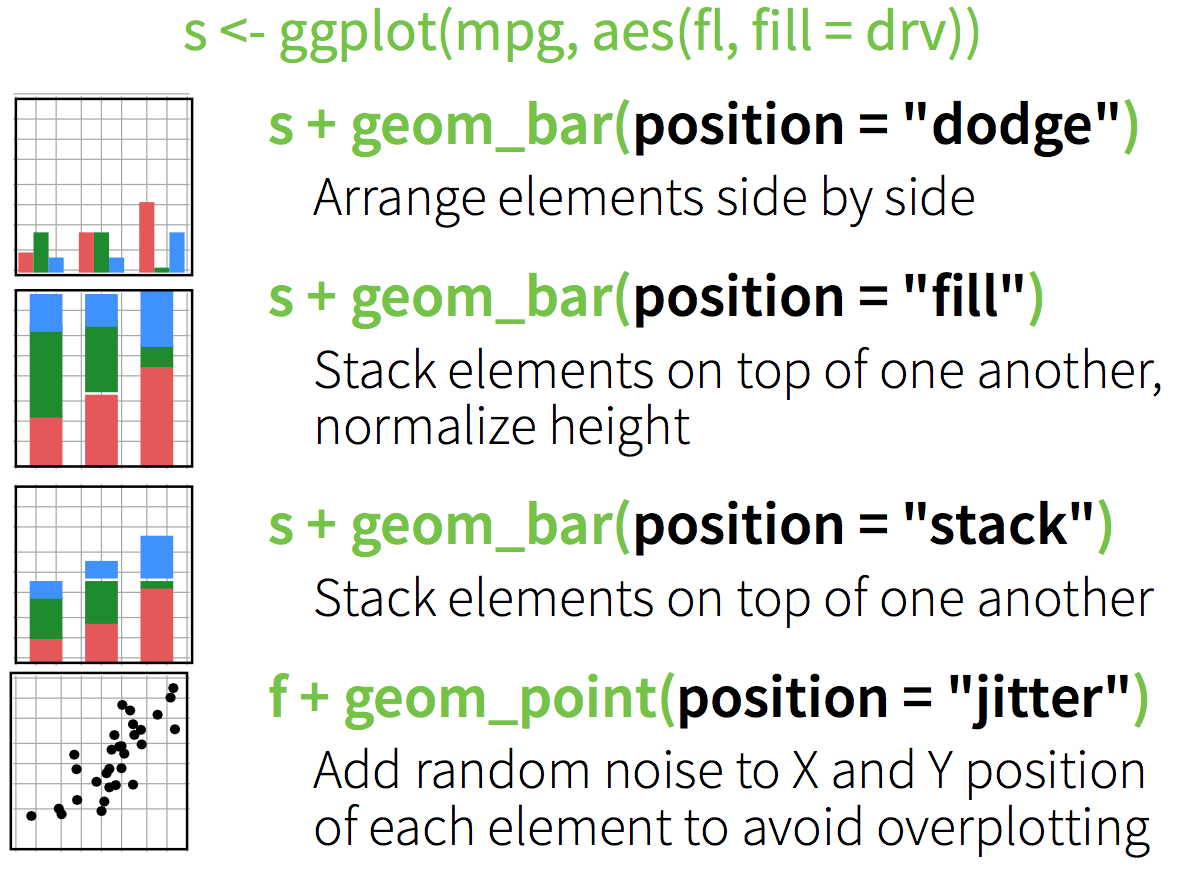### 2.10.1 Stacked bars

``ggplot(diamonds, aes(clarity, fill=cut)) + geom_bar()``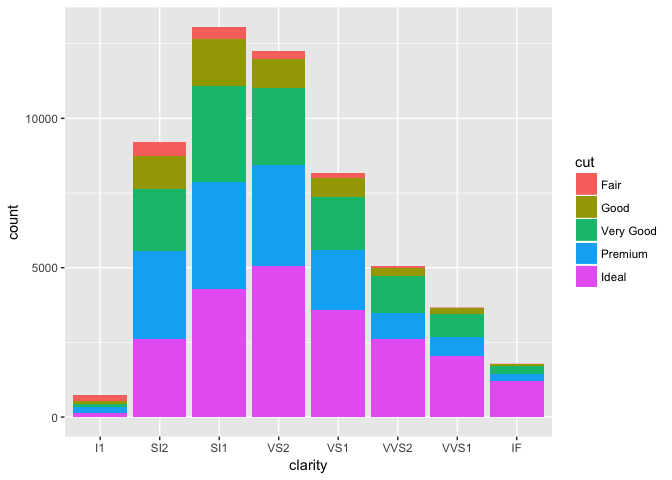### 2.10.2 Dodged bars

``ggplot(diamonds, aes(clarity, fill=cut)) + geom_bar(position="dodge")``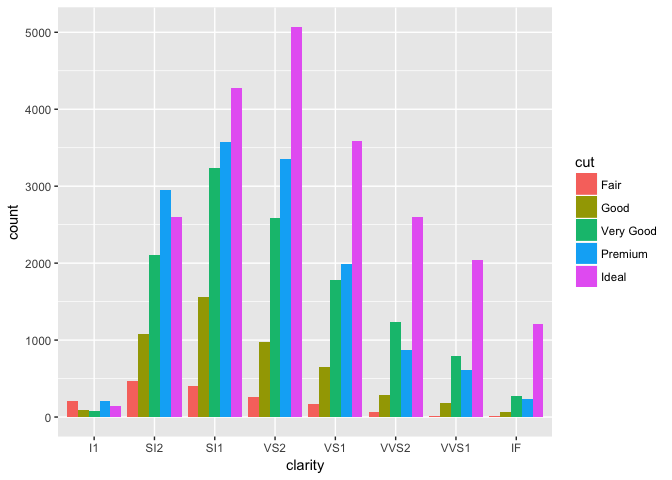# 3 Facets

Use facets to divide graphic into small multiples based on a categorical variable.

`facet_wrap()` for one variable:

``````ggplot(mpg, aes(x = cty, y = hwy, color = factor(cyl))) +
geom_point()+
facet_wrap(~year)``````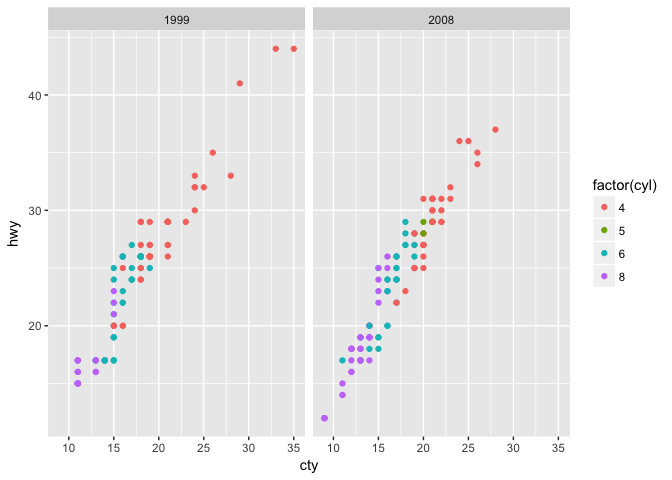`facet_grid()`: two variables

``````ggplot(mpg, aes(x = cty, y = hwy, color = factor(cyl))) +
geom_point()+
facet_grid(year~cyl)``````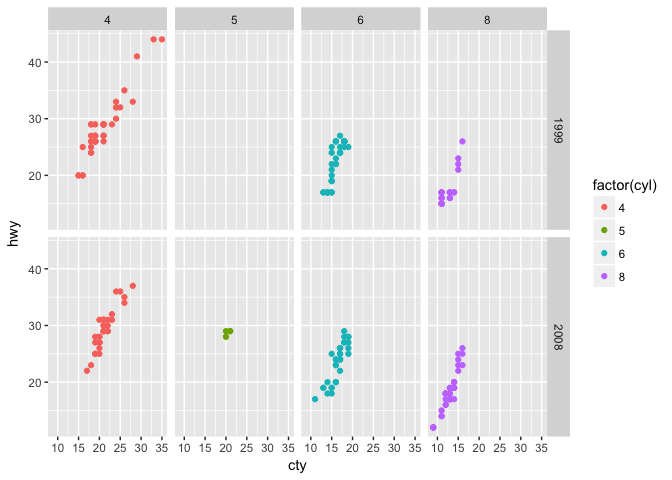Small multiples (via facets) are very useful for visualization of timeseries (and especially timeseries of spatial data.)

# 4 Themes

Set default display parameters (colors, font sizes, etc.) for different purposes (for example print vs. presentation) using themes.

## 4.1 GGplot Themes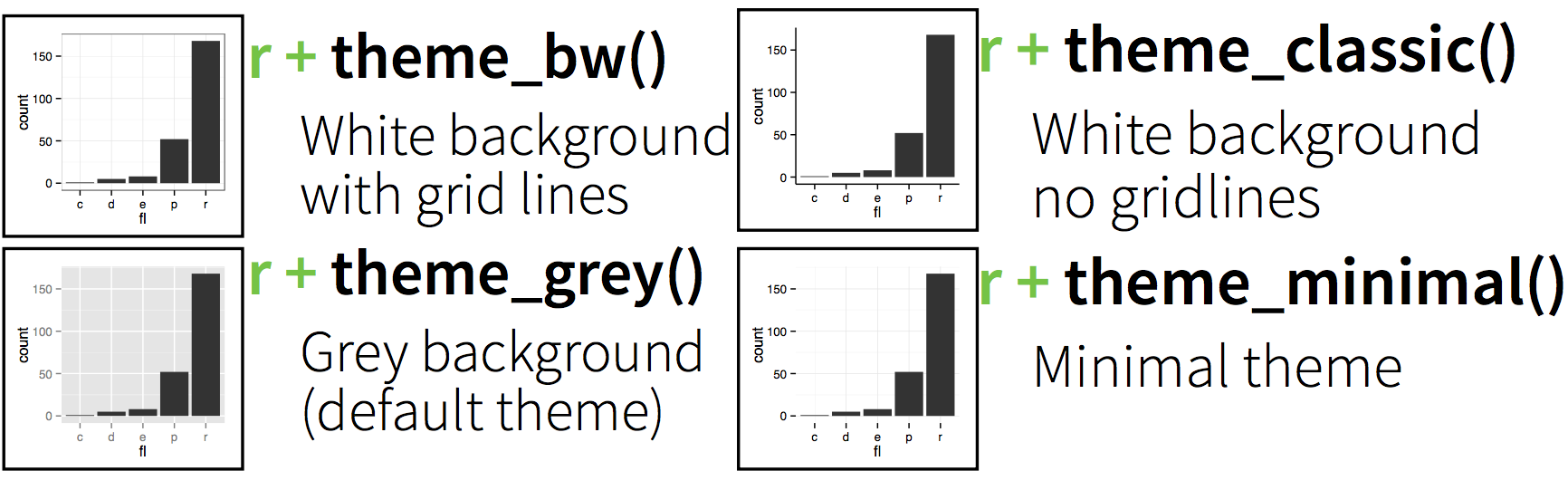Quickly change plot appearance with themes.

### 4.1.1 More options in the `ggthemes` package.

``library(ggthemes)``

### 4.1.2 Theme examples: default

``````p=ggplot(mpg, aes(x = cty, y = hwy, color = factor(cyl))) +
geom_jitter() +
labs(
x = "City mileage/gallon",
y = "Highway mileage/gallon",
color = "Cylinders"
)``````

### 4.1.3 Theme examples: default

``p``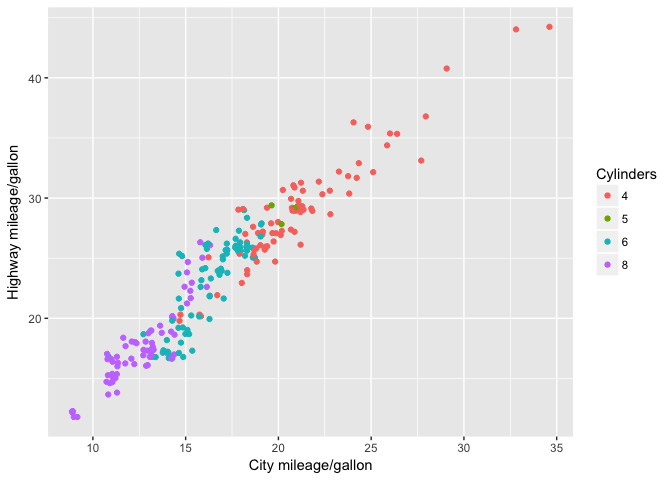### 4.1.4 Theme examples: Solarized

``p + theme_solarized()``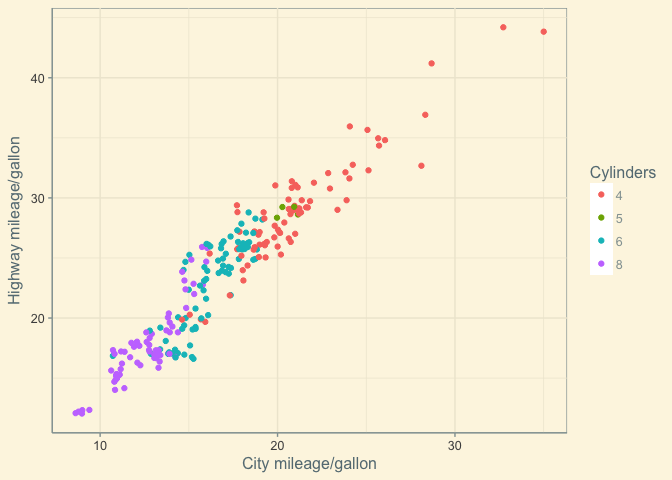### 4.1.5 Theme examples: Solarized Dark

``p +  theme_solarized(light=FALSE)``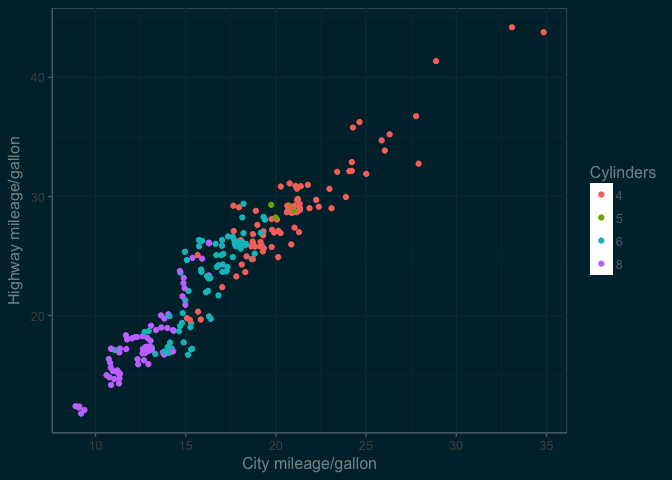### 4.1.6 Theme examples: Excel

``p + theme_excel() ``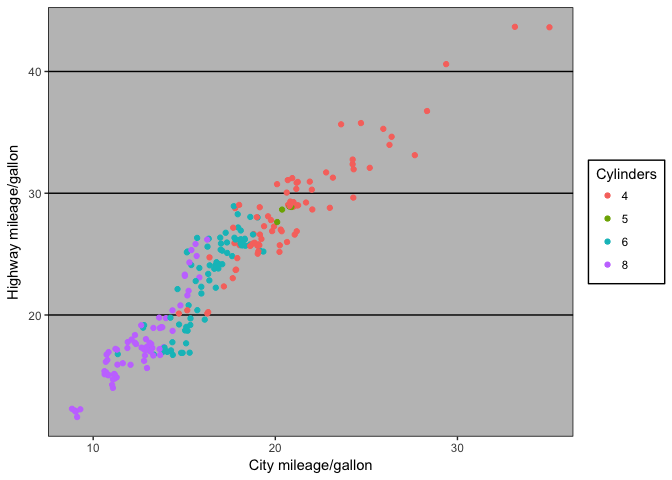### 4.1.7 Theme examples: The Economist

``p + theme_economist()``
``````## Warning: `panel.margin` is deprecated. Please use
``````## Warning: `legend.margin` must be specified
## using `margin()`. For the old behavior use
## legend.spacing``````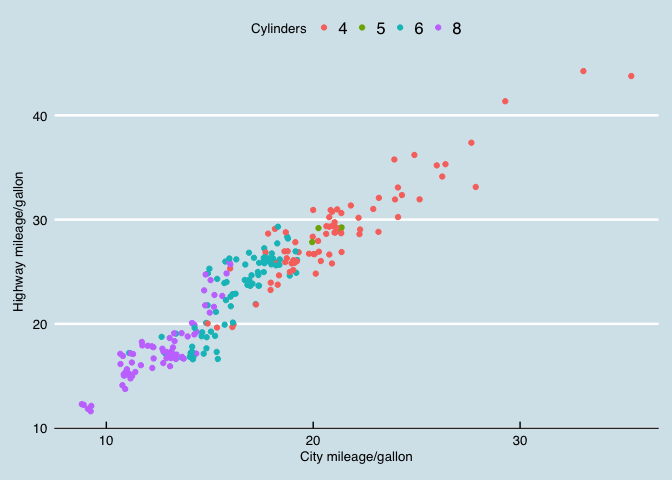# 5 Saving/exporting

## 5.1 Saving using the GUI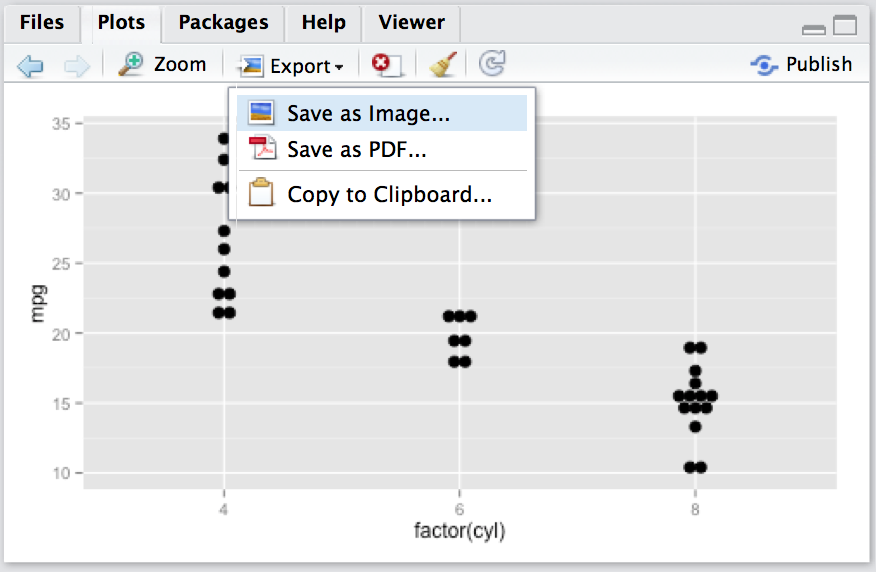## 5.2 Saving using `ggsave()`

Save a `ggplot` with sensible defaults:

``ggsave(filename, plot = last_plot(), scale = 1, width, height)``

## 5.3 Saving using devices

Save any plot with maximum flexibility:

``````pdf(filename, width, height)  # open device
ggplot()                      # draw the plot(s)
dev.off()                     # close the device``````

Formats

• pdf
• jpeg
• png
• tif

and more…

1. Save the `p` plot from above using `png()` and `dev.off()`
2. Switch to the solarized theme with `light=FALSE`
3. Adjust fontsize with `base_size` in the theme `+ theme_solarized(base_size=24)`

## 5.3 Save a plot: Example 1

``````png("03_assets/test1.png",width=600,height=300)
p +  theme_solarized(light=FALSE)
dev.off()``````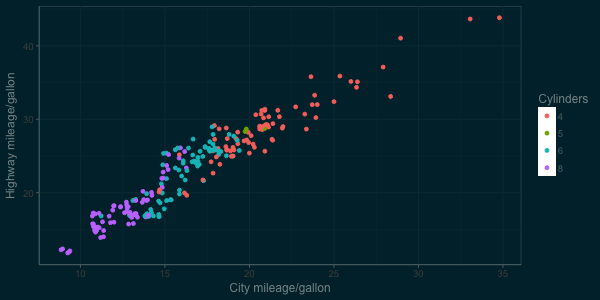## 5.3 Save a plot: Example 2

``````png("03_assets/test2.png",width=600,height=300)
p +  theme_solarized(light=FALSE, base_size=24)
dev.off()``````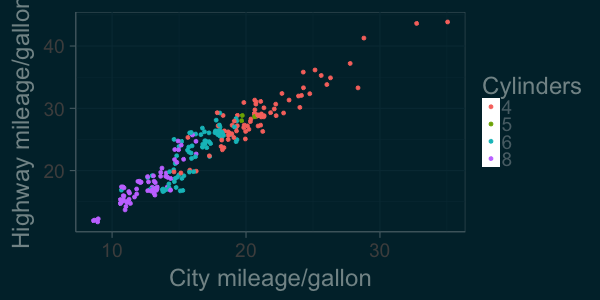## 5.4 GGPLOT2 Documentation

Perhaps R’s best documented package: docs.ggplot2.org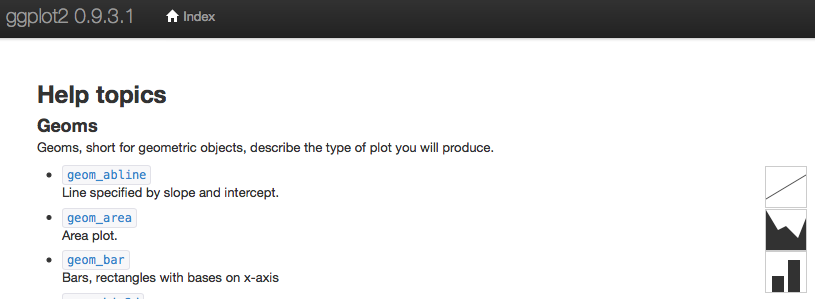Sources:

Licensing: